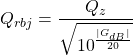# Quantisation of Poles in the Z-plane

Back in this posting, I talked about biquads and their use in digital signal processing for making linear filters (what most of us call “equalisers”). Part of that explanation showed that a biquad is formed of a feed-forward section and a feed-back section, and you could swap the order of these two and get the same results. In a “Direct Form 1” implementation, the feed-forward comes first, as shown in Figure 1.

in the “Direct Form 2” implementation, the feed-back comes first, as shown in Figure 2.

There are advantages and disadvantages to each of these implementations, depending on things like how the rest of your system is implemented, and what, exactly you’re expecting the biquad to do.

However, for this posting, we’re going to “zoom in” a bit to the feed-back portion of the above diagrams. This portion of the biquad provides the “poles” in the Z-plane, as I described in the “Intuitive Z-plane” series of postings last week.

If I separate the feed-back portion of the above figures, it would look like Figure 3:

The locations of the poles (and therefore the magnitude response) of this portion of the filter are dependent on the gains at the outputs of the two 1-sample delays. What happens when these gains do not have an infinite resolution (which they can’t, because everything in a digitally-represented world is quantised to a finite number of steps)?

Before we go any further with this, I have to put in a reminder that quantisation (or “rounding”) in a DSP world is done in binary – not decimal. So, the quantisation that I’m about to do isn’t the same as stopping a couple of digits after the decimal…

In a world with infinite resolution, I can set the values of those two gains to be anything I want, and therefore the poles in my system can be anywhere within the circle. (We’ll assume that we don’t want a pole on the circle because that would result in a gain of ∞ dB, which is very loud.) However, let’s say that we quantise the gain values to a 4-bit binary value, where the first bit is reserved for the +/- indicator. This means that we only have +/- 2^3 possibilities, or 8 values, one of which is +0, and another is -0 (which is the same thing…). So, in other words, we have 7 possible negative values, 7 possible positive values, and 0.

The end result of this is that the poles have a limited number of locations where they can be placed. For the 4-bit quantisation described above, the resulting locations look like Figure 4.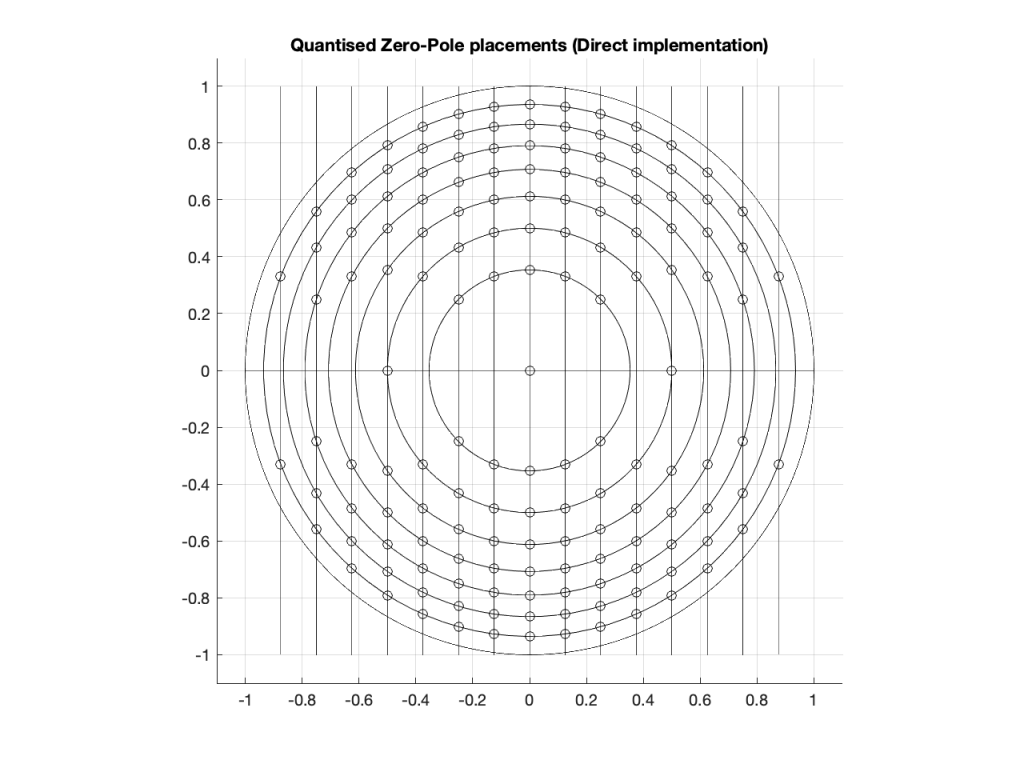Figure 4: The circles indicate the possible locations of the poles as a result of quantisation of the gain coefficients in a 4-bit Direct Form implementation.

Remember that we’re not talking about quantisation of the audio signal – it’s quantisation of the gain coefficients inside the biquad that will have an impact on the response of the filter.

Of course, it would be crazy to implement a biquad using only 4-bit quantisation for the gain coefficients. However, the point of this posting is not to show that biquads suck. It’s only to show one possibly important aspect of them if you’re a DSP engineer – but we’re getting there.

Just for fun, let’s increase the resolution of the system to 6 bits: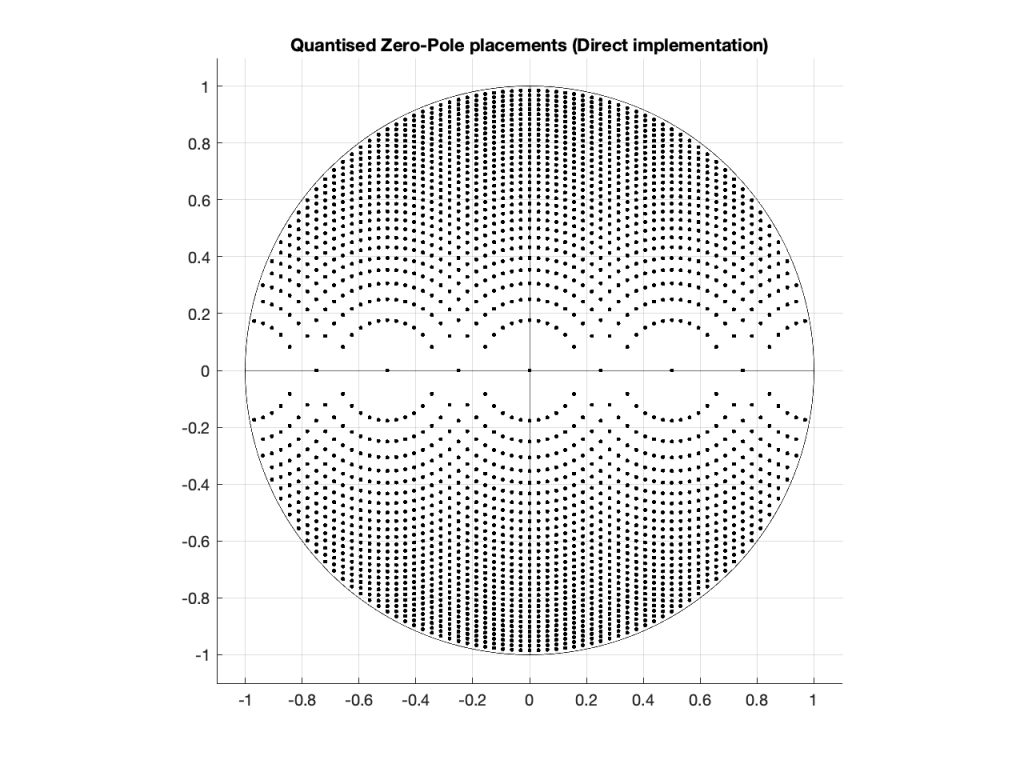Figure 5: The dots indicate the possible locations of the poles as a result of quantisation of the gain coefficients in a 6-bit Direct Form implementation.

… or to 8 bits: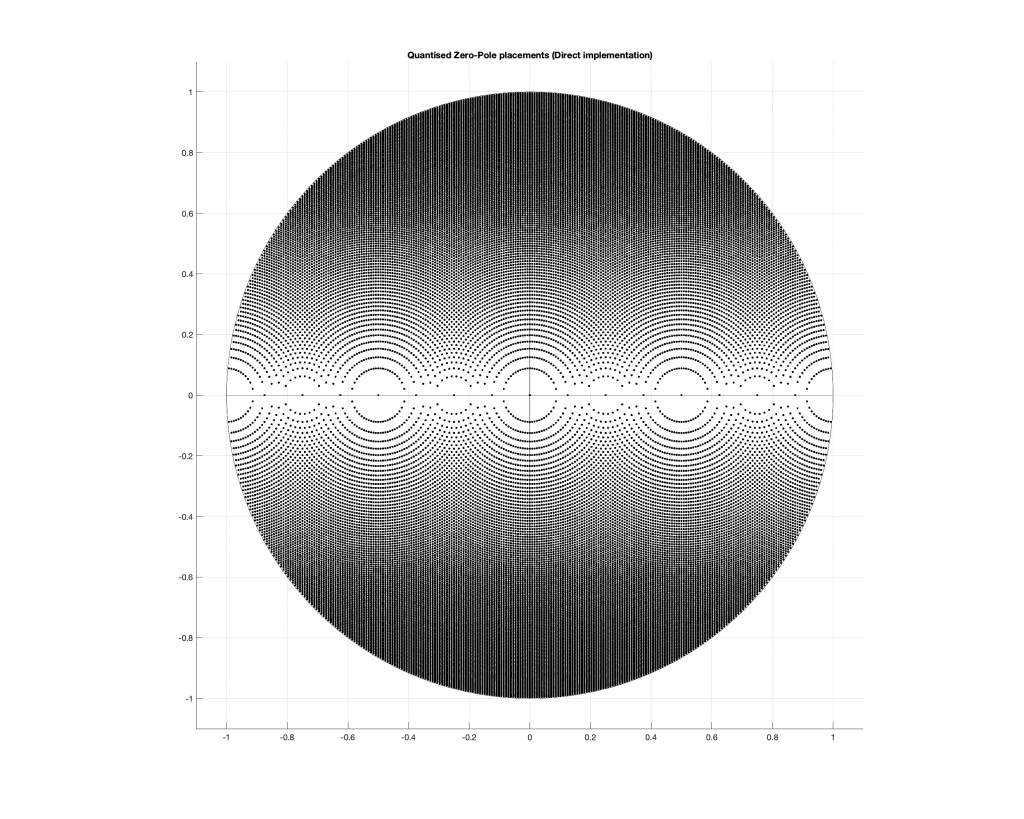Figure 6: The dots indicate the possible locations of the poles as a result of quantisation of the gain coefficients in a 4-bit Direct Form implementation.

Any higher than this and the pattern will just get so dense that it’ll turn black, so I’ll stop.

## So what?

At this point, you may be asking “so what?” The answer to this very important question lies on the far right side of all of those graphs. Notice that there aren’t any locations near the point on the graph where X=1 and Y=0. You may remember from Figure 7 in this posting that I did last week, that that’s the point on the Z-plane that corresponds to very low frequencies.

This means that, if you want to create a digital filter, and you need a pole near 0 Hz, you’re going to run into some trouble with a Direct Form implementation of the biquad. Yes, the higher the bit depth of the gain coefficients, the closer you can get, but this might not be the best way to do things.

There is another option for implementing your feed-back portion of the biquad. You can use a design called a Coupled Form instead, which is shown in Figure 7 (compare it to Figure 3).

Notice that you still have two 1-sample delays, however there are now 4 gains instead of 2. How can this be better? Well, in a system with infinite resolution on the gain coefficients, it’s not. Given the appropriate choice of gain values, this implementation will do exactly the same thing as the Direct Form implementation if your resolution is infinite.

However, if you have a limited resolution, then the available locations of the poles on the Z-plane are very different. Let’s use the 4-bit, 6-bit, and 8-bit quantisations of the gain values again: these are shown in Figures 8 to 10.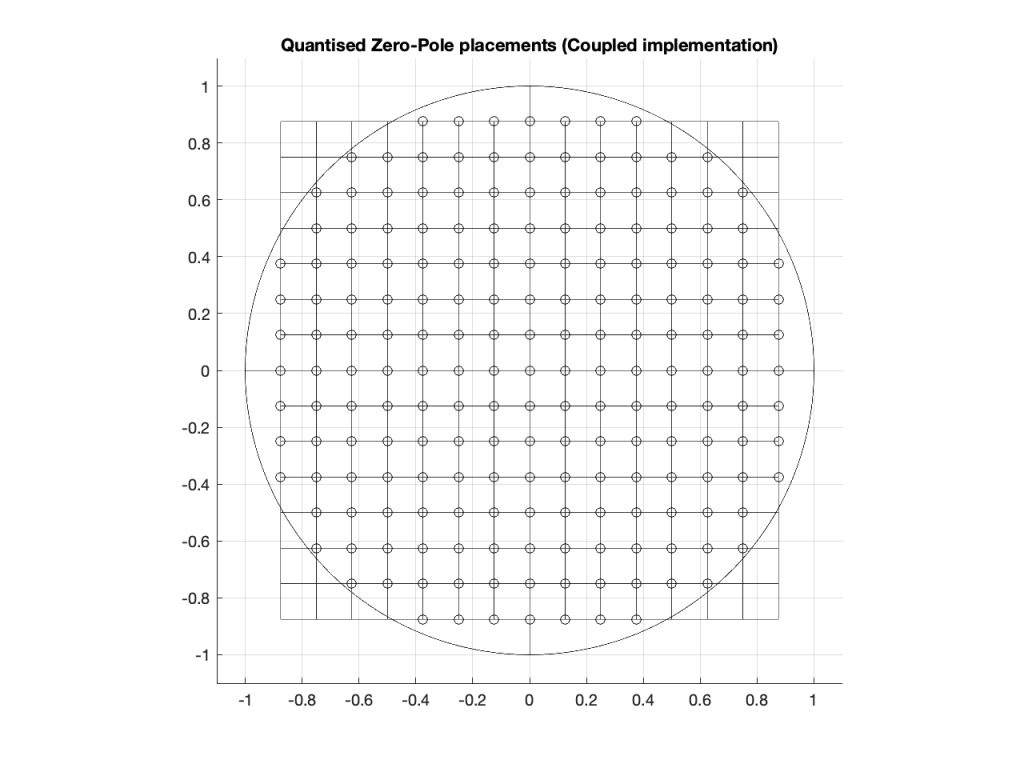Figure 8: The circles indicate the possible locations of the poles as a result of quantisation of the gain coefficients in a 4-bit Coupled Form implementation.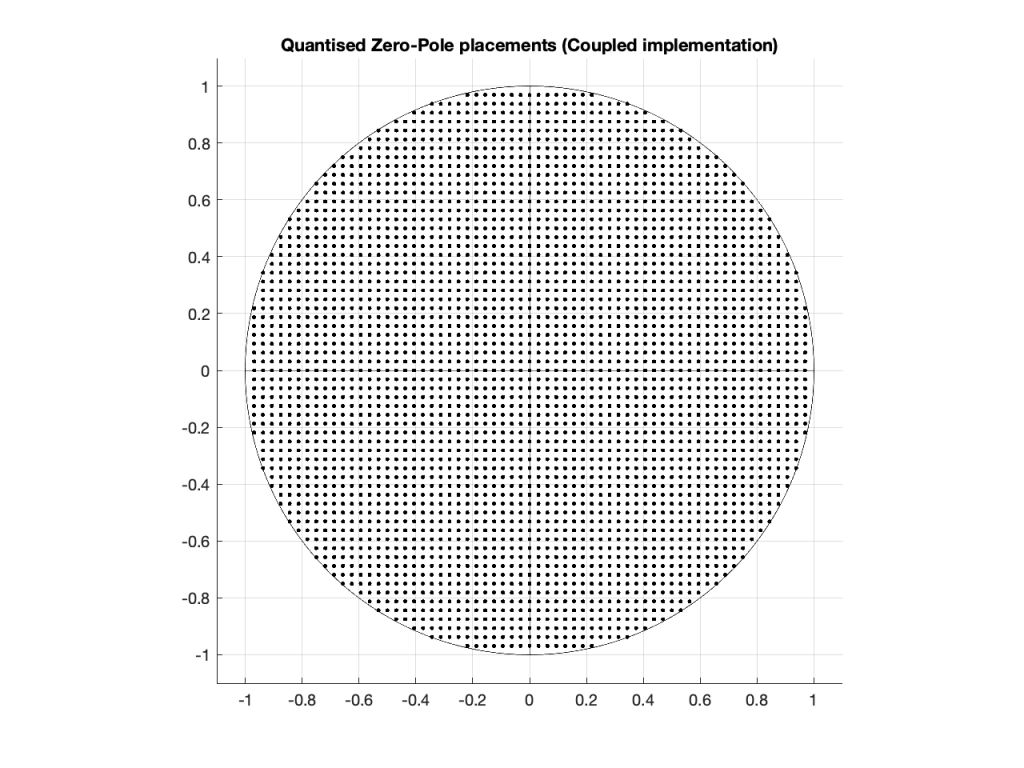Figure 9: The dots indicate the possible locations of the poles as a result of quantisation of the gain coefficients in a 6-bit Coupled Form implementation.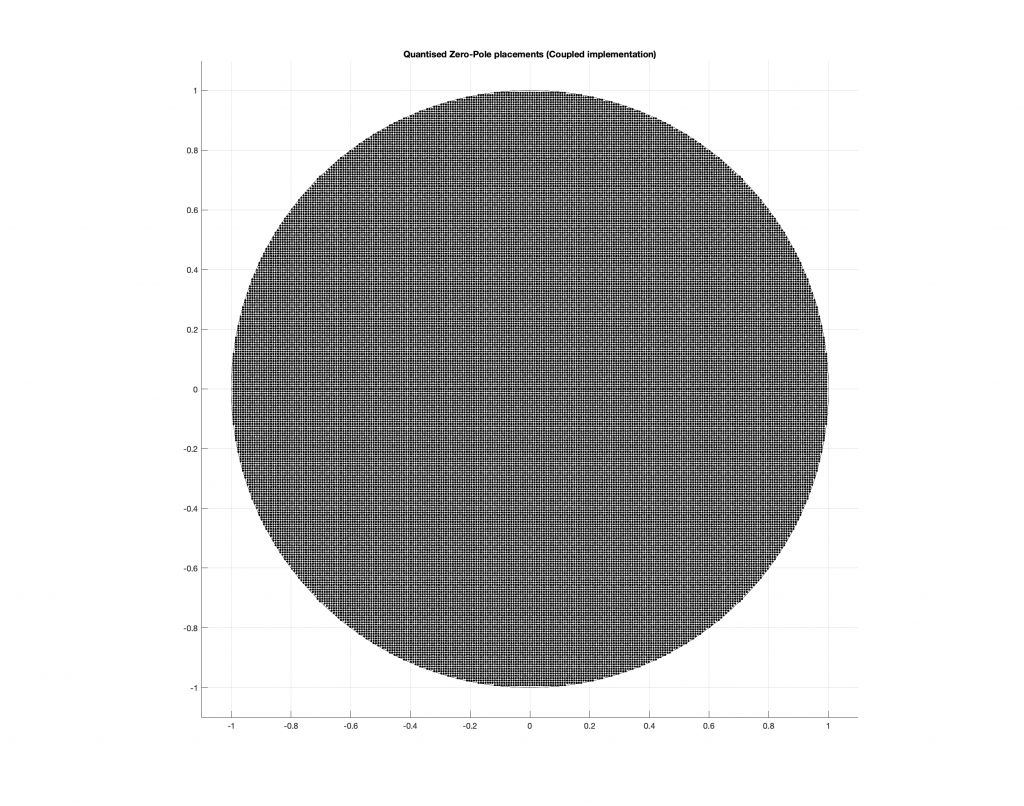Figure 10: The dots indicate the possible locations of the poles as a result of quantisation of the gain coefficients in a 8-bit Direct Form implementation.

As you can see in Figures 8 through 10, the Coupled Form implementation, given the same resolution on the gain coefficients, will give you much better placement opportunities for the poles in the low frequency region than the Direct Form implementation.

Of course, in all of these examples, I’m only showing up to an 8-bit word, and a typical DSP runs uses a lot more than 8 bits for the gain coefficients. So, it’s possible that, in the real world, the actual resolution is high enough that this is of no concern whatsoever.

However, if you’re building a very-low-frequency filter an if the magnitude response isn’t exactly what you’re looking for, this might help you get a little closer to your goal.

It’s important to point out here that the quantisation of the locations of the zeros is not the same as this. Someday, I’ll come back to this and plot the expected vs. actual magnitude responses of some biquads where I’ve quantised the zeros and poles, just to see how badly things go wrong when they do…

This posting is a simple summary of the discussion of a section called “Poles of Quantized Second-Order Sections” in “Discrete-Time Signal Processing” by Alan V. Oppenheim and Ronald W. Schafer.

The Coupled Form implementation was introduced by C.M. Rader and B. Gold in their paper called “Digital Filter Design Techniques in the Frequency Domain” from the Proceesings of the IEEE, Vol. 55, pp. 149 – 171 , from February, 1967.

# Intuitive Z-plane: Part 5 – Conclusion

If you’ve read through the first four parts of this series, then you’re already at a point where you can intuitively understand what’s going on. We just have a couple of details to take care of before finishing off.

Firstly, the plots showing the zeros and poles in the figures you’ve been looking at plots of the “Z-plane” or “Complex-plane“. As I said at the start, we’re only trying to get to an intuitive understanding of these plots – so I’m not going to get into complex numbers, or even much math (apart from what you’ll see below… which isn’t very complicated, and avoids complex numbers).

When I’m developing a new DSP algorithm, I use an application called Max from cycling74.com. Figure 1 shows a screenshot from Max, where I’m using an object to calculate the biquad coefficients to make a low pass filter, as you can see. I’ve then connected the output of that object (it looks like a magnitude response) to a Z-plan representation that shows me the same thing in a different way.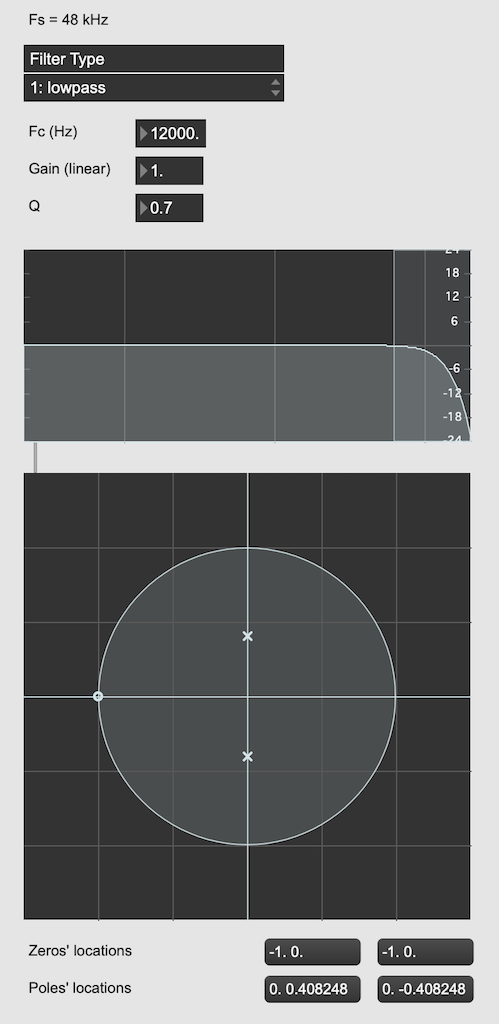Figure 1: The top plot shows the magnitude response of the filter. The bottom plot shows the Z-plane representation of the same filter.

You may notice that this plot has two poles, one at (0, 0.408) and the other at (0, -0.408). In fact there are two zeros there as well, but they’re situated in the same place, on “on top” of the other, at (-1, 0). This is always true for a biquad – there are always two zeros and two poles. Sometimes, they’re located in the same place, sometimes not, sometimes they’re placed symmetrically, sometimes not, depending on the filter, as we’ll see below.

Let’s look at that Z-plane representation in 3-dimensions: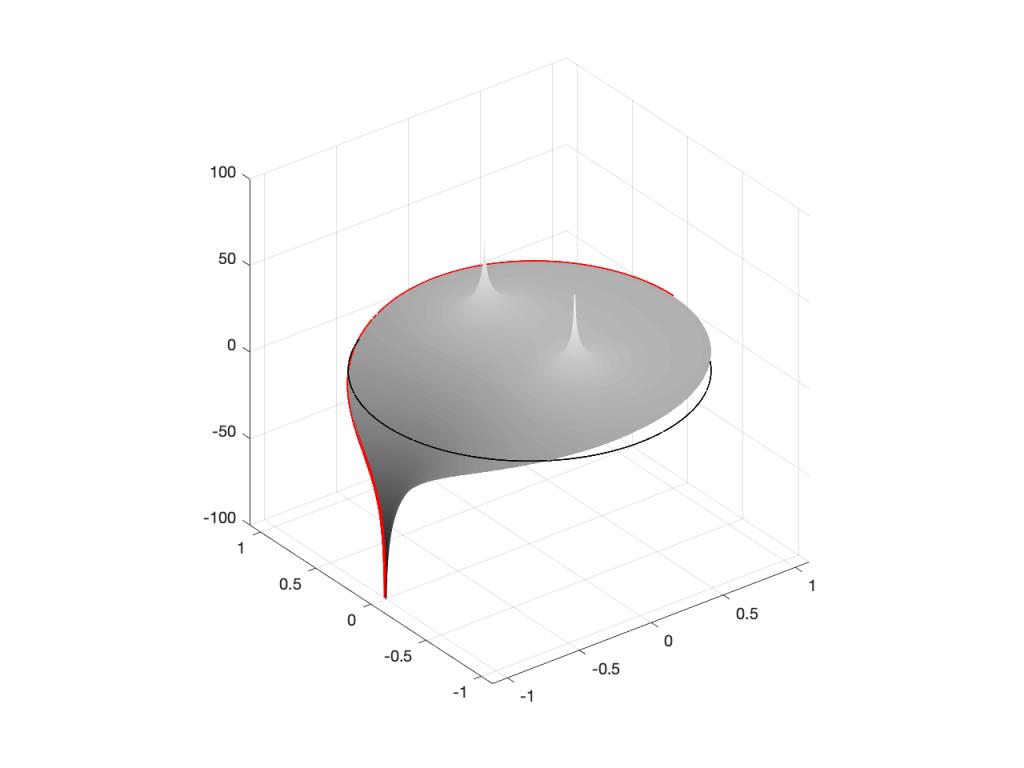Figure 2: A 3D view of the Z-plane representation of the filter shown in Figure 1.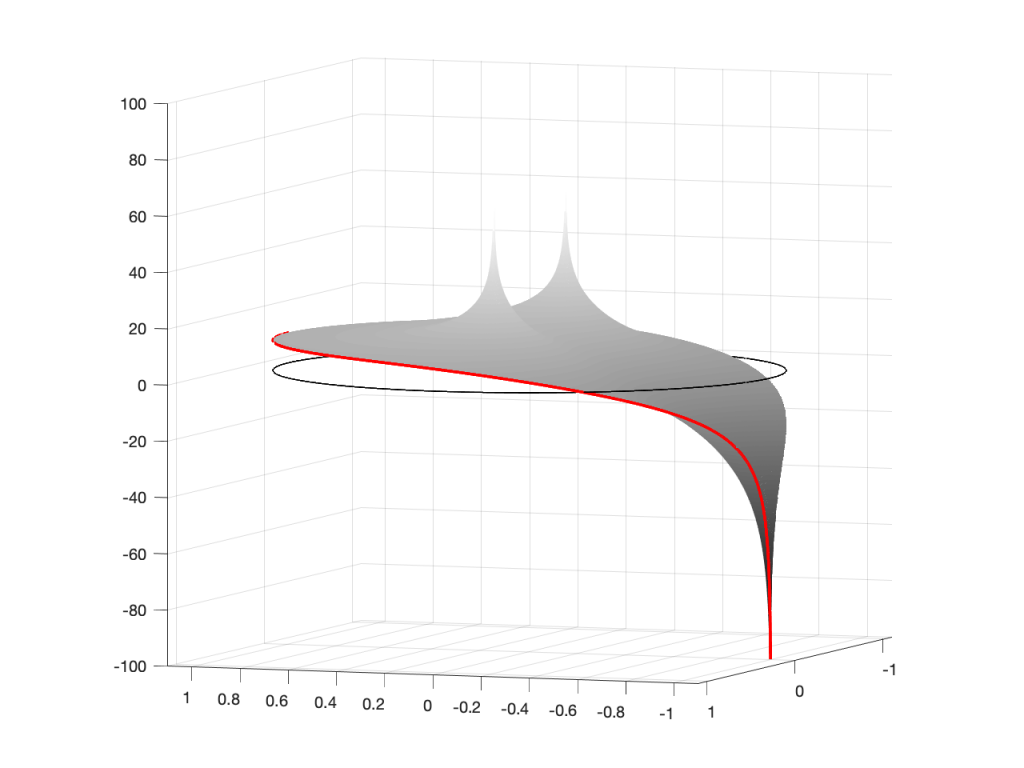Figure 3: The same plot as shown in Figure 2, rotated to show the back of the plot.

So, as you would now expect, the poles pull up the edge of the circle, and the zeros (both in the same place) pull down, giving the red line the height that it has.

Now, think back to this Figure from earlier in the series:

If you therefore look at Figure 3, which is like looking at Figure 4 from the top, you’ll notice that the height of the red line (the edge of the circle is high on the left (in the low frequencies) and drops as you go to the right (the high frequencies). This is the magnitude response that’s shown on the top of Figure 1. The only difference is that it’s on a linear scale instead of a logarithmic scale, so the shape looks a little weird.

Let’s do another one: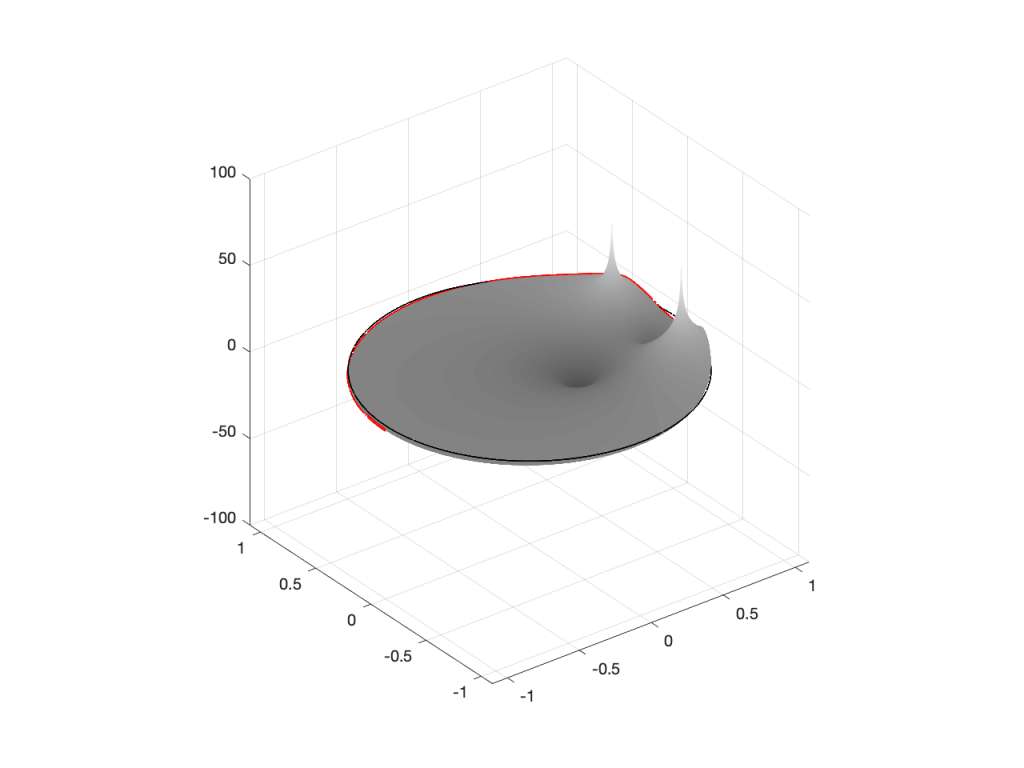Figure 6: A 3D view of the Z-plane representation of the filter shown in Figure 5.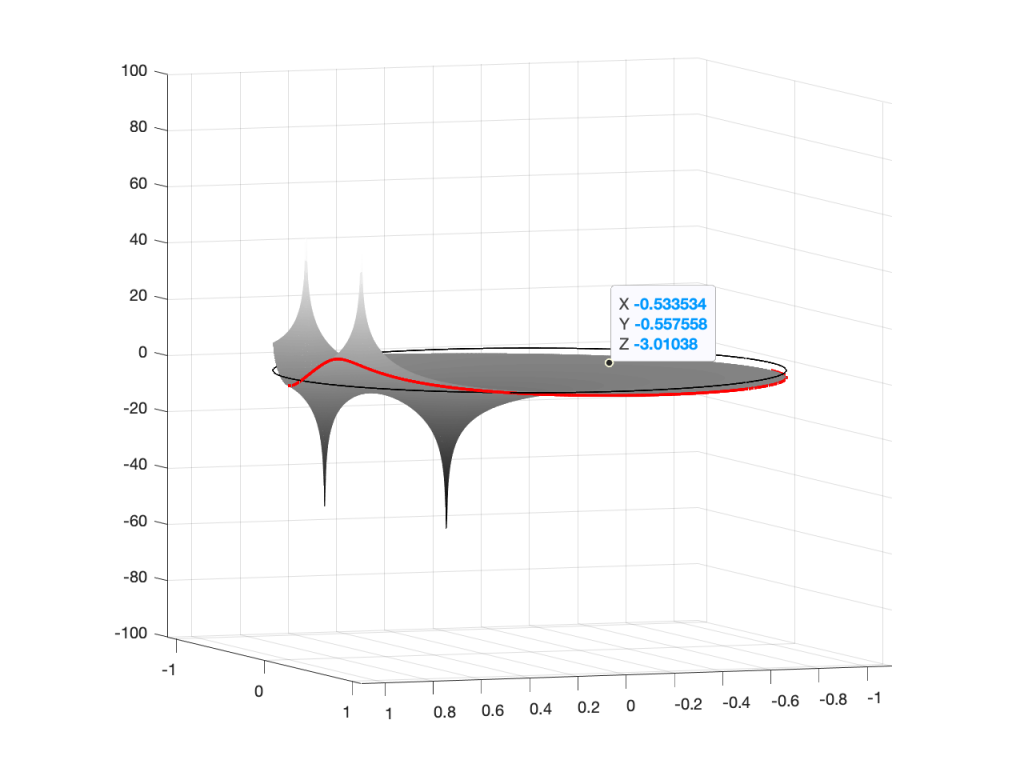Figure 7: The same plot as shown in Figure 6, rotated to show the back of the plot.

Hopefully, now you are able to look at a Z-plane representation of a filter and think about the effect of the poles and zeros on the edge of the circle, and therefore get a rough idea of the magnitude response of the filter…

If not, I apologize for wasting your time. On the other hand, if you’re in a life-threatening situation, this knowledge probably wouldn’t help you anyway… Very few people have gotten a critical injury in a biquad accident.

## How I did it

If you want to make these plots for yourself, the math is pretty simple.

Start by choosing the frequency, which will be a point on the circle. You then find the four distances from the zeros and poles to that point (I’ve indicated those distances in Figure 8 with the variables z1, z2, p1, and p2.) This can be done using the Pythagorean theorem.

To find the gain of the filter at the frequency, you divide the sum of the zeros’ distances by the sum of the poles’ distances. In other words:

(z1 + z2) / (p1 + p2)

That will give you the result as a linear value. If you then want to convert it to decibels, like I’ve done, you do a little extra math like this:

20 * log10 ( (z1 + z2) / (p1 + p2) )

That’s it! You just need to do repeat that math for each frequency that you’re interested in, and you’re done!

# Intuitive Z-plane: Part 4 – How, not what

I ended Part 3 by saying that DSP engineers think of the frequency scale as a circle rather than as a straight line. The questions are “Why do they think like this? What’s wrong with them?” Although I can’t answer the second question, the answer to the first question is fundamentally simple.

A DSP engineer is not really interested in what a filter does. She or he is interested in how it filters. A normal magnitude response plot shows us mortals the result of what’s happened to the audio after it’s gone through a filter (or a processor in general). Someone making that filter (or system) needs to know how it’s working instead.

So far, in this series, we’ve seen the following:

• Digital audio filters are made with feed-forward and feed-back delays with different gains.
• Feed-forward delays make narrow dips (and wide peaks) in the magnitude response
• Feed-back delays make narrow peaks (and wide dips) in the magnitude response
• DSP engineers think of frequency on a circle instead of a straight line
• DSP engineers also want to see plots of how a filter works instead of its result on the audio signal.

Let’s put all of this together.

We’ll draw the circle showing the frequency scale, but then rotate the view to see it in three dimensions. For example, I can pretend to make the surface of of the circle out of a rubber sheet that can be pulled upwards (like a tent) or downwards (like a funnel), whilst always maintaining a circular edge.

If I want to pull the tent upwards, I’ll use a “pole” to do it. That pole has an infinite height (we’re going to need some very stretchy rubber). If I want to pull the funnel downwards, I’ll use something I’ll call a “zero“. (I am not going to go into why zeros and poles are called that, so as to avoid doing too much math.)

So, if I were to put a zero in the middle of the circle, its 2D representation would look like Figure 1 (notice the red circle in the middle showing where the zero is placed), and the 3D version would look like Figure 2: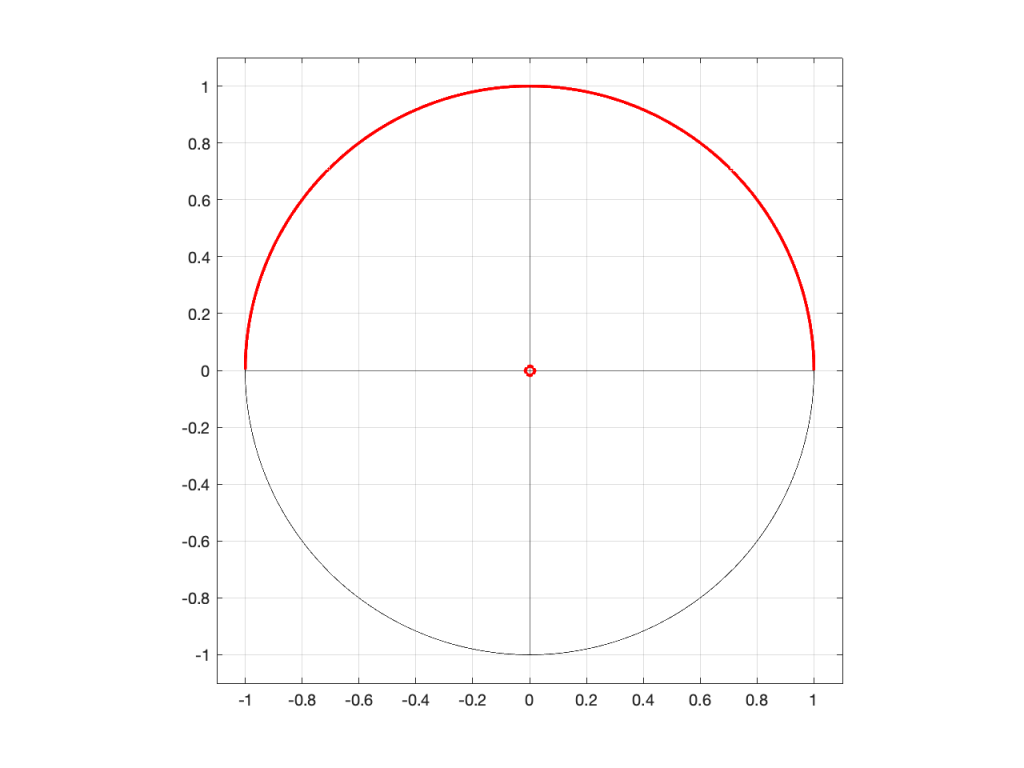Figure 1: A 2D representation of a “zero” (indicated by a red circle) in the middle of the circle that shows the frequency scale (the red line shows the scale going counter-clockwise from 0 Hz on the right to Fs/2 on the left)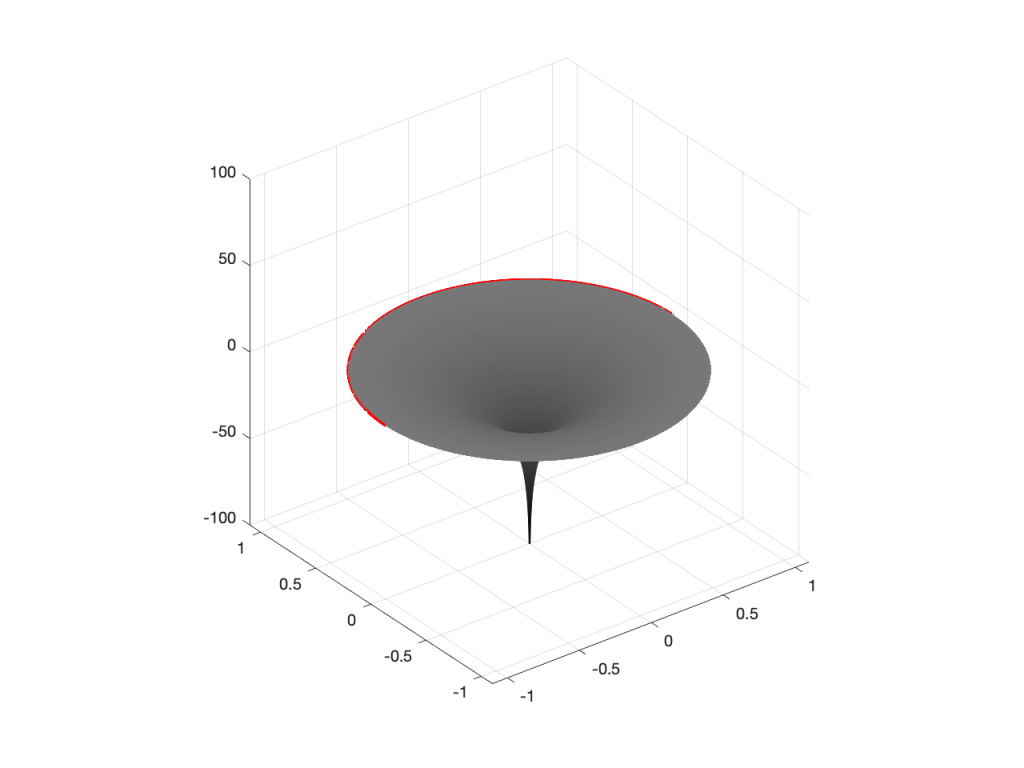Figure 2: A 3D representation of the same information shown in Figure 1.

If I were to put the pole (indicated by a red ‘x’) in the middle of the circle instead, then the result would look like Figures 3 and 4.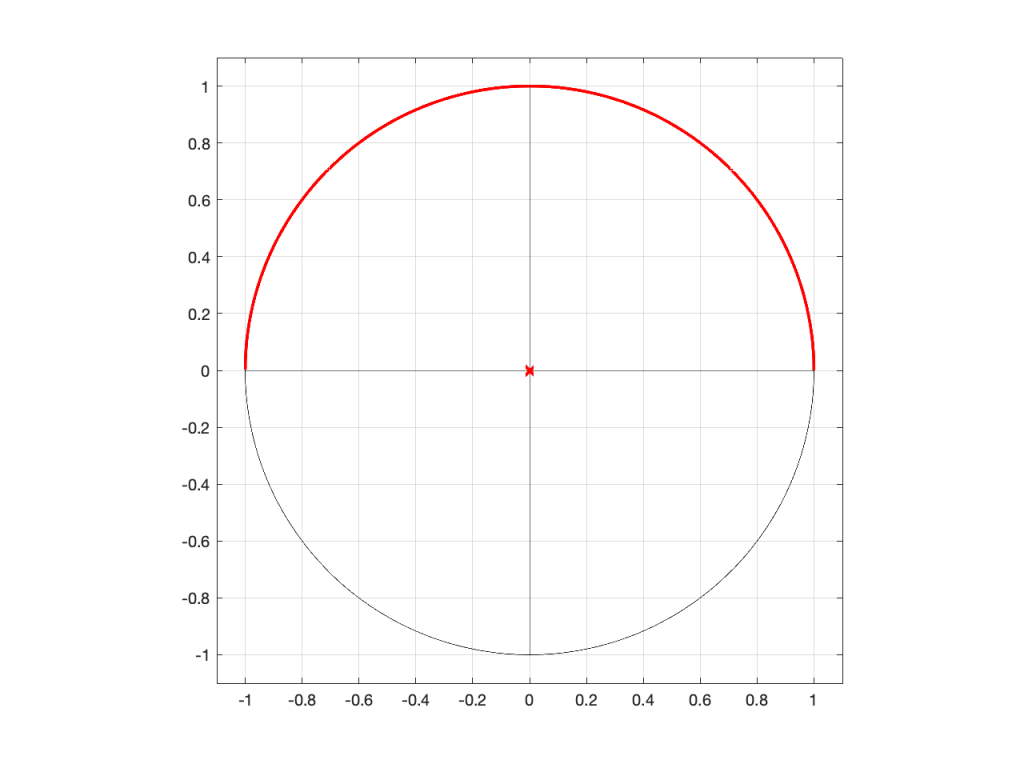Figure 3: A 2D representation of a “pole” (indicated by a red circle) in the middle of the circle that shows the frequency scale (the red line shows the scale going counter-clockwise from 0 Hz on the right to Fs/2 on the left)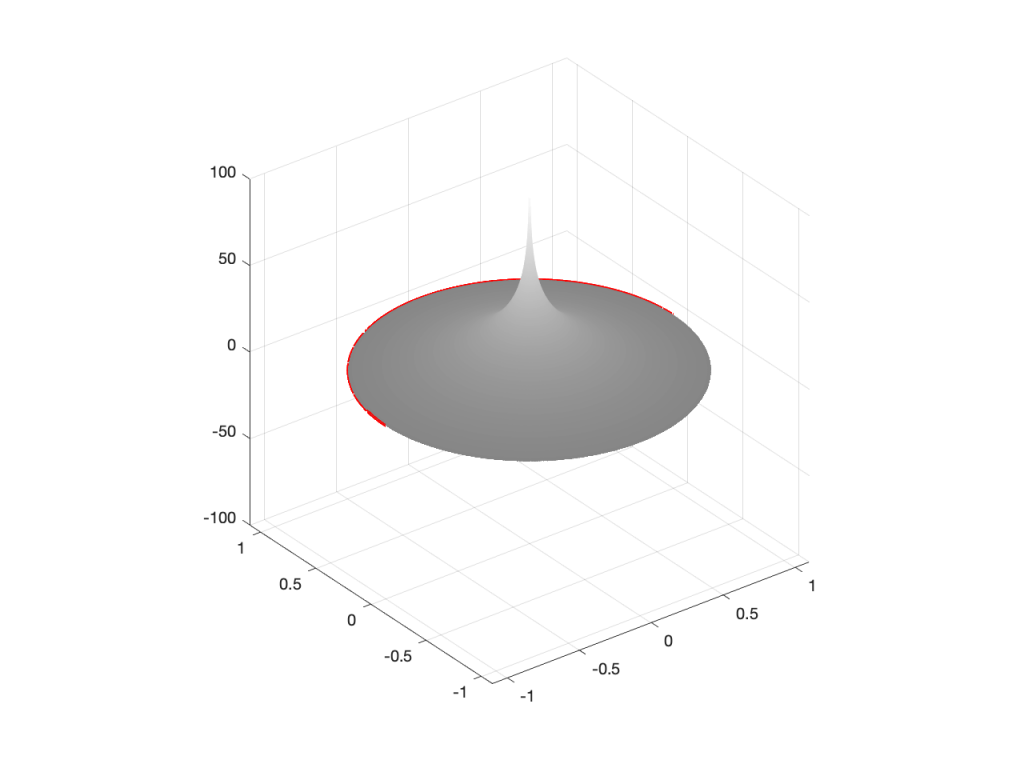Figure 4: A 3D representation of the same information shown in Figure 3.

So far so good… If we were to rotate Figures 2 or 4 and look at the red line that I’ve drawn around the edge, we’d see that it’s flat with a height of 0 (on the vertical scale) all the way around. This is because I’ve carefully placed the zero or the pole at the exact middle of the circle, so it’s pulling equally on all points of the edge of the “tent” or the “funnel”.

However, what would happen if I moved the zero or the pole away from the middle? Some examples of this for a zero moved to the location (-0.75, 0) are shown in Figures 5 to 7, below.

As you can see in Figure 7, when the zero is moved away from the centre of the circle, it pulls downwards on the closer edge (notice how the red line is lower than the black line which has a constant height of 0). However, it also doesn’t pull downwards as much on the opposite side of the circle (notice how the red line is higher than the black line on the left side).

Of course, if I were to do the same thing with a pole, everything would behave symmetrically, as shown in Figures 8 to 10.

We’re almost finished… One more posting to go to wrap up.

# Intuitive Z-plane: Part 1 – Introduction

Back in this posting, I made the following statement:

Generally speaking, digital filters work by taking an audio signal, delaying it, changing the level, and adding the result back to the signal itself.

I then showed an example of a simple digital filter like this:

If we use the filter in Figure 1, set the delay to 0, and set the gain to 1, then the output is just the input signal added to itself, so it’s two times the amplitude or about 6 dB louder.

If we leave the gain at 1, and set the delay to something else – let’s say, 1 ms, for example – then, in the very low frequencies (say, 1 Hz) the phase difference caused by the 1 ms delay is almost nothing – therefore the output will be +6 dB. At 500 Hz, however, the 1 ms delay is equal to a 180º phase shift, so the output of the delay will always be opposite in polarity with the non-delayed signal. Therefore, at 500 Hz, this filter will have no output. At 1 kHz, the output will be +6 dB again, because 1 ms = 360º at 1 kHz. At 1.5 kHz, there’s no output (540º phase shift), at 2 kHz, we’re back to +6 dB, and so on all the way up. The result is a magnitude response that looks like this: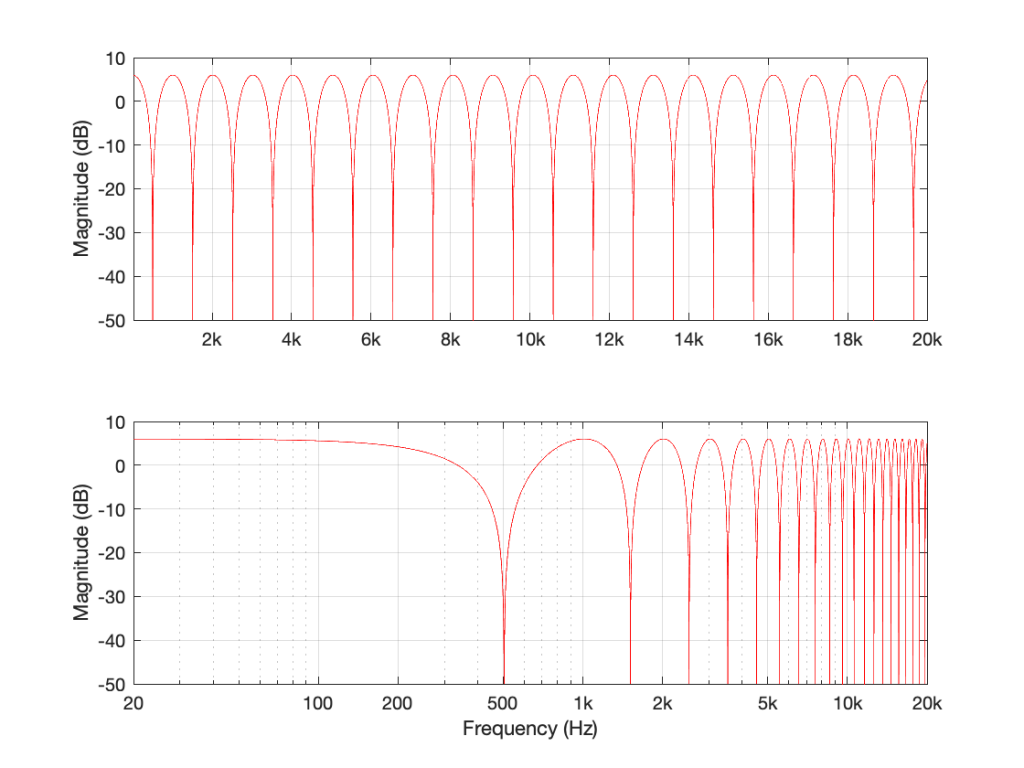Figure 2: The magnitude response of the filter shown in Figure 1, if Delay = 1 ms and Gain = 1. Note that these are two identical plots, the only difference is the scaling of the X-axes.

If I used the same delay time on the same filter structure, but set the gain to something between 0 and 1 – let’s make it 0.75, for example, then the overall shape would be the same, but the effect would be less, as shown in Figure 3.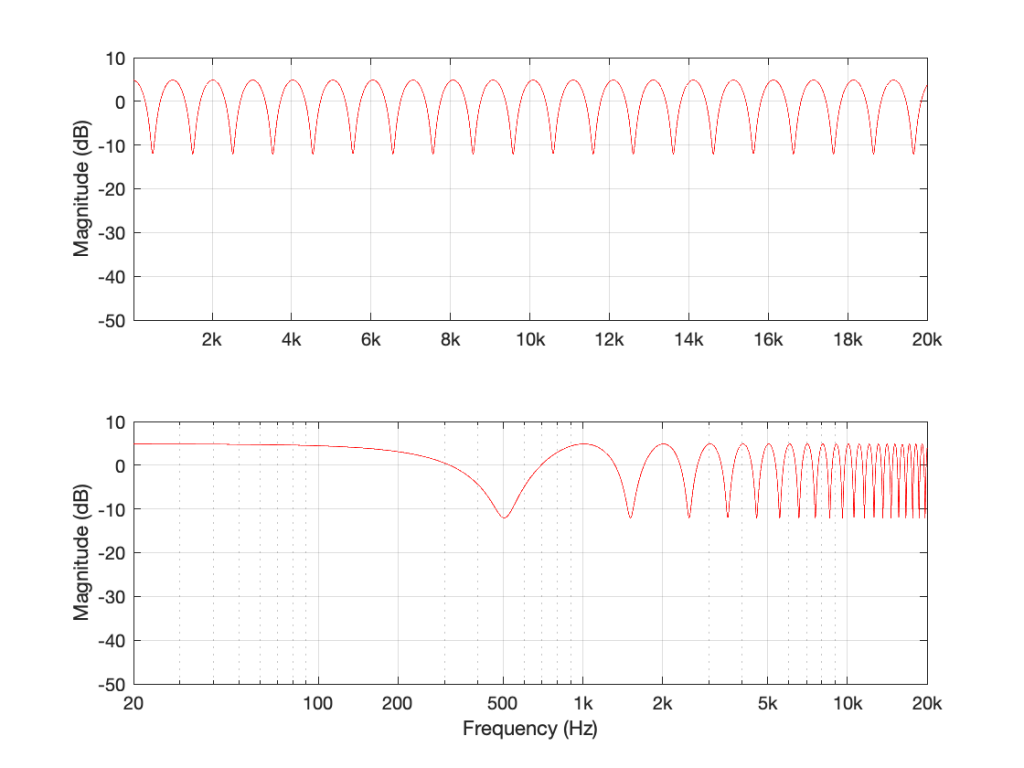Figure 3: The magnitude response of the filter shown in Figure 1, if Delay = 1 ms and Gain = 0.75.

If we make the gain a negative value, then the overall shape remains the same, but the high points and the low points swap places because the delayed signal is now cancelling where it was adding, and vice versa.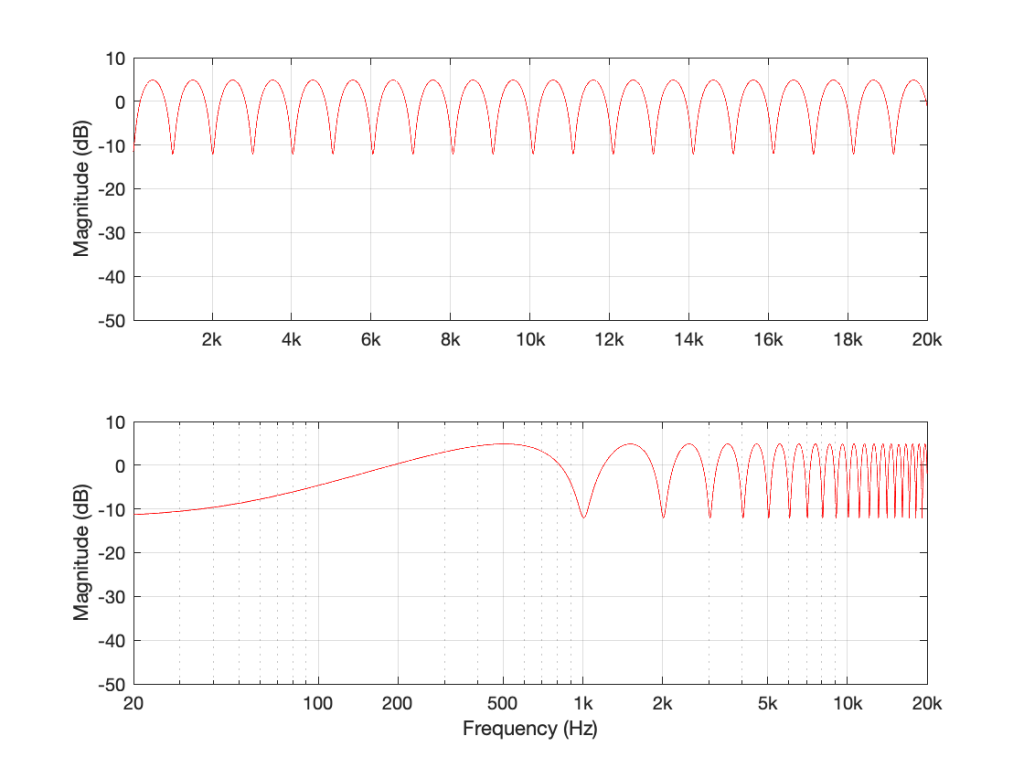Figure 4: The magnitude response of the filter shown in Figure 1, if Delay = 1 ms and Gain = -0.75. Notice how the boosts and dips have swapped places as a result of using a negative gain.

Let’s think about this intuitively. If my audio signal cannot exceed a value of 1 (which is normally the way we work… a full-scale signal ranges from -1 to 1) and the gain of the delay output in the filter in Figure 1 also ranges from -1 to 1, then the maximum possible output of the filter is 2.

If I had two delay lines and I were summing all three signals (the input and the two delayed signals) and the gains were still limited within the range of -1 to 1, then the maximum possible output would be 3…

However, the minimum possible output level (not the minimum possible output value) would be no signal (as in the case of a 500 Hz input in Figure 2. This is equivalent to an output of -∞ dB.

If I generalise this, then I can say that if your filter is built using ONLY the summed outputs of feed-forward delays, then the maximum possible output can be easily calculated, and the minimum possible output is no signal.

Still generalising: notice that the “bumps” in the above frequency responses are smooth and rounded, and the dips are pointy notches.

What happens if the filter uses feed-back instead of feed-forward?

Let’s set the delay time to 1 ms again, and set the gain to 0.99. I chose 0.99 instead of 1 because this means that each time the signal re-circulates back, it will get a little bit quieter. If I had set the gain to 1, then the delay would keep “echoing” forever. If I made it greater than 1, then the output would get louder on each re-circulation, and things would get very loud, sooner or later…

So, if Delay = 1 ms and Gain = 0.99 in the filter in Figure 5, the resulting magnitude response looks like Figure 6.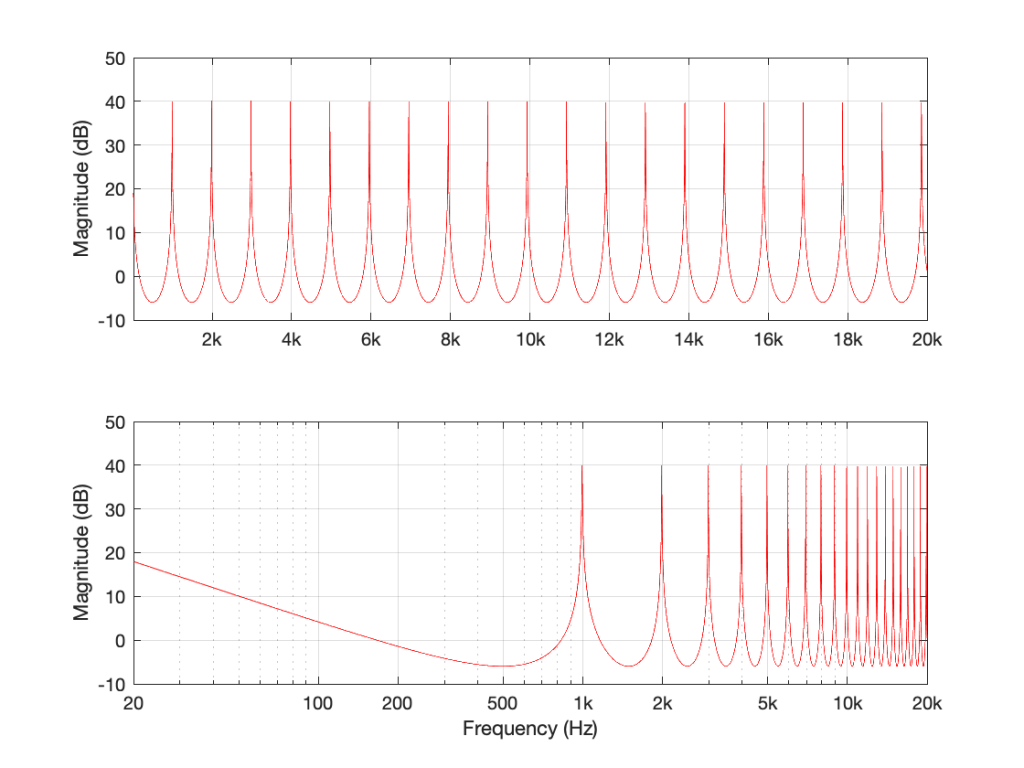Figure 6: The magnitude response of the filter in Figure 5 when Delay = 1 ms and Gain = 0.99.

There are some things to notice in Figure 6.

Firstly, notice that the overall “shape” of the curve is upside-down relative to the one in Figure 2. The rounded bits are on the bottom and the pointy bits are on the top. This means that instead of having very narrow notches, you have very narrow resonances that are “singing” like a collection of sinusoidal waves, one at the frequency of each peak.

Secondly, notice that the peaks and the dips are in the same places as in Figure 2. In both cases, the Delay = 1 ms and the gain is positive, so the frequencies that are boosted are the same in both cases. For example, both have a peak at 1 kHz, and a dip at 500 Hz.

Thirdly, notice that the overall level is much, much higher. 40 dB is a LOT louder than 6 dB – this is because the sum of all those re-circulated signals echoing over and over in the filter add up to something loud over time.

If I reduce the gain, but keep it positive, then (just like in the case with the feed-forward filter) the shape of the magnitude response stays the same, it’s just reduced in effect.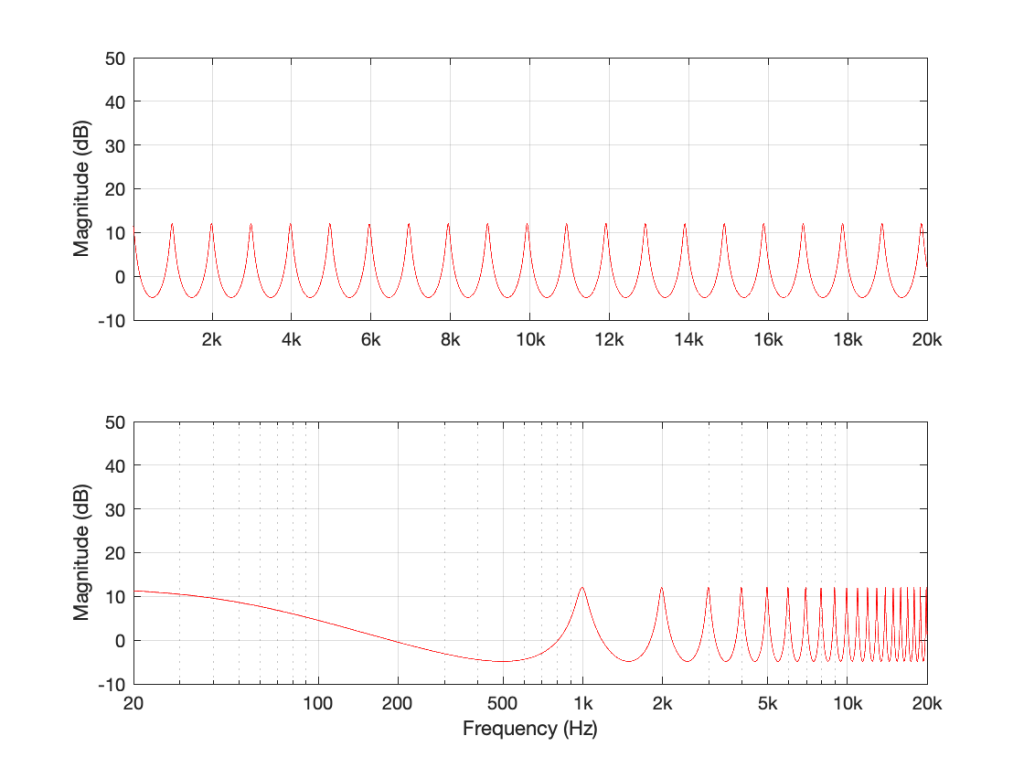Figure 7: Delay = 1 ms and Gain = 0.75. Notice that the peaks are smaller because the lower gain causes the signal to “die away” faster.

If I did the same thing, but set the gain to a negative number (say, -0.99) instead, then each time the signal re-circulates, it flips polarity. The resulting magnitude response looks like Figure 8.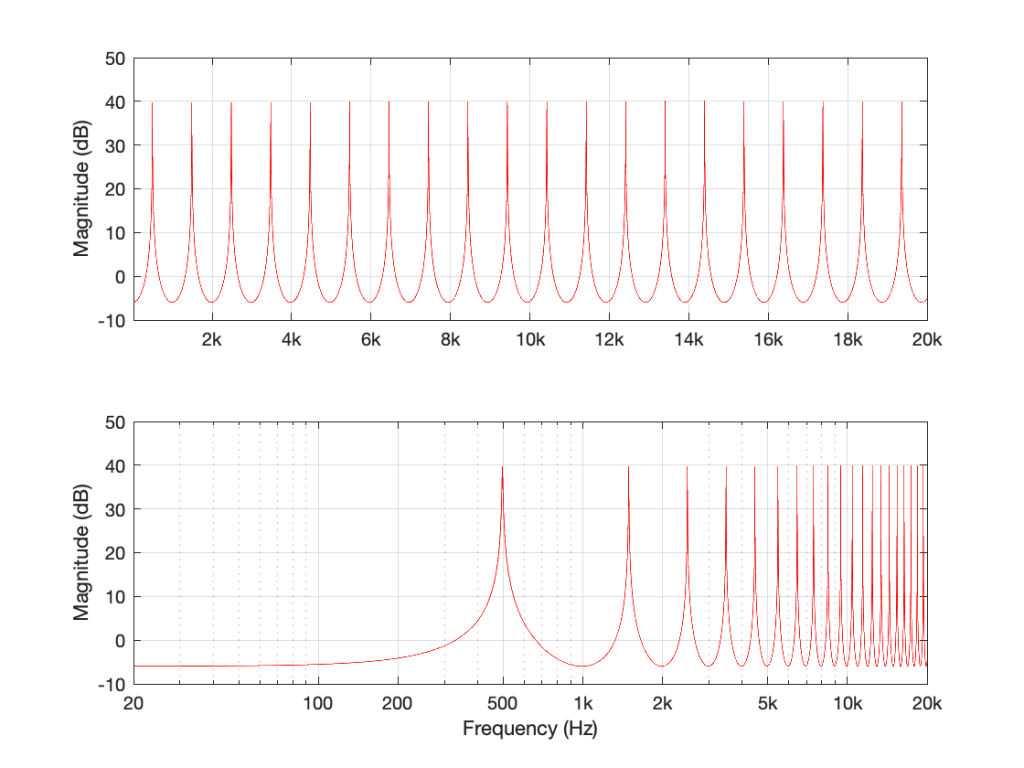Figure 8: The filter in Figure 5 where Delay = 1 ms and Gain = -0.99.

Notice that this is related to the magnitude response in Figure 4 – we have less output in the low end, and now the first peak is at 500 Hz instead of 1 kHz.

If I generalise this one, then I can say that if your filter is built using ONLY the summed outputs of feed-back delays, then the peaks are much higher than with a feed-forward design because they’re resonating.

Still generalising: notice that the “bumps” in the above frequency responses are pointy (because they’re resonances), and the dips are smooth and rounded.

## The summary (for now)

Repeating myself, because this is the take-away information for this posting:

• If your filter is built using ONLY the summed outputs of feed-forward delays, then:
• the maximum possible output can be easily calculated
• the minimum possible output is no signal
• the “bumps” in the above frequency responses are smooth and rounded
• the dips are pointy notches.
• if your filter is built using ONLY the summed outputs of feed-back delays, then:
• the peaks are much higher in level than with a feed-forward design because they’re resonating
• the “bumps” in the above frequency responses are pointy because they’re resonating
• the dips are smooth and rounded

# “High-Res” Audio: Part 4 – Know your limits

If you’ve read the three introductory parts of this series, linked above; and if you’re still awake, then we are ready to start putting things together and jumping to incorrect conclusions…

Let’s say that you’ve been hired to specify a digital audio system for some reason (we’ll assume that it’s an LPCM system – nothing exotic). Using the information I’ve told you so far, you can make two decisions in your specification:

You select a bit depth to be enough to give you the dynamic range you desire. In this case, “dynamic range” means the “distance” in level between the loudest sound you can record / store / transmit (I isn’t say what the “digital audio system” was going to be used for) and the inherent noise floor of the system. If you’re recording the background noise on an airplane while it’s in flight, you don’t need a big dynamic range, because it’s always loud, and never changes. However, if you’re recording a Japanese Taiko Drummer group, you’ll need a huge usable dynamic range because the loud parts of the performance are a LOT louder than the quietest parts.

As we saw in Part 3, an LPCM digital audio system cannot record any audio that has a frequency higher than 1/2 the sampling rate. So, you select a sampling rate that is at least 2x the highest frequency you’re interested in. For example, if you believe the books that say you can hear from 20 Hz to 20,000 Hz, then you might decide that your sampling rate has to be at least 40,000 Hz. On the other hand, if you’re making a subwoofer that you know will never be fed a signal above 120 Hz, then you don’t need a sampling rate higher than 240 Hz.

Don’t get angry yet. I’m just keeping these numbers simple to make the math easy. Later on, I’ll explain why what I just said might not be correct.

## Mistake #1

I just jumped to at least three conclusions (probably more) that are going to haunt me.

The first was that my “digital audio system” was something like the following:

As you can see there, I took an analogue audio signal, converted it to digital, and then converted it back to analogue. Maybe I transmitted it or stored it in the part that says “digital audio”.

However, the important, and very probably incorrect assumption here is that I did nothing to the signal. No volume control, no bass and treble adjustments… nothing.

## Mistake #2

We assumed above that we can define the system’s dynamic range based on the dynamic range of the audio signal itself. However, this makes the assumption that the noise floor of the digital system and the noise floor of your audio signal are identical, which is probably not true. As we saw in Part 2, the noise generated by TPDF dither is white – it has the same probability of having a given amount of energy per Hertz. Since we hear sound logarithmically (meaning that, to us, octaves are equal widths. Equal spacings in Hz are not.) This means that the noise sound “bright” to us – because there’s just as much energy in the top octave (say, 10 kHz to 20 kHz, if you believe the books) as there is in all other frequencies combined from 0 Hz up to 10 kHz.

If, however, the noise floor in your concert hall where the taiko drummers are playing is caused by the air conditioning system, then this noise will be a lot louder in the low frequencies than the the highs – which is not the same.

Therefore it’s too simplistic to say “the noise floor of the digital system” and the “noise floor of the signal” – since these two noise floors are different. (As Steven Wright said: “It doesn’t matter what temperature the room is, it’s always room temperature.”)

## Mistake #3

As we’ll see later, if you’re going to do anything to the signal while it’s in the “digital domain”, then you need to take that into consideration when you’re deciding on your sampling rate. It’s not enough to say “useful audio bandwidth times 2” because there are some side effects that need to be remembered…

However, counter-intuitively, it could be that, in order to improve your system, you’ll want to make the sampling rate LOWER instead of HIGHER – so this is not a simple case of “more is better”.

We’ll get to that topic later. For now, I’ll leave you in suspense.

## Some details

One thing we saw in Part 3 was that, if we have an audio signal with energy at a frequency higher than 1/2 the sampling rate, and if that signal gets into the analogue-to-digital converter (ADC), then the output of the ADC will contain an error. We’ll get out energy at frequencies that were not in the original, due to the effect called “aliasing“.

Once that’s in the digital audio signal, there’s no removing it, so we need to make sure that the too-high-frequency signals don’t get into the ADC’s input in the first place. This is done using a low-pass filter that (in theory) removes all energy in the signal above the Nyquist frequency (which is equal to 1/2 the sampling rate). Since that low-pass filter prevents aliasing, we call it an anti-aliasing filter. Normally, these days, that antialiasing filter is built into the ADC itself.

As we also saw in Part 3, the digital-to-analogue converter (DAC) has to smooth out the digital signal to convert it from a “staircase” wave to a smoother one. That’s also done with a low-pass filter that eliminates all the harmonics that would be required to make the staircase have sharp corners. Since this is done to re-construct the analogue signal, it’s called a “reconstruction filter“.

This means that, if we pull apart some of the components in the signal chain I showed in Figure 1, it really looks more like this:

On to Part 5.

# “High-Res” Audio: Part 1

I’ve been debating writing a series of postings about “high resolution” audio for a long time – years. Lately, (probably because of some hype generated by some recent press releases) I’ve been getting lots of question (no, that’s not a typo) about it, so it appears the time has come…

To start: the question that I get (a lot) is “If I can’t hear above 20 kHz, then what’s the use of high-res?” As I’ll explain as we go through, this is only one, rather small aspect to consider in this topic. In fact, it might be the least important issue to consider.

However, before I write too much, I’ll say that I’m not going to argue for or against higher resolutions in digital audio systems. I’m only going to go through a bunch of issues that can be used to argue either for or against them. So, there’s not going to be a big reveal at the end of this series telling you that high-res is either better, worse, or no different than whatever you’re using now. It’s merely going to be a discussion of a number of issues that need to be weighed. The problem is that this entire topic is complicated – and there’s no single “right” answer, as I’ll argue as we go along.

To start, let’s get down to basics and look (once again, from the perspectives of this website) at what sound is, and how it’s converted from an analogue electrical signal into a digital representation. The good thing is that I’ve written this introduction before in a different series of postings. So, I’m going to be extremely lazy and just copy-and-paste that information here. I’m not just referring you to another page because I’m intentionally leaving some things out because we’re headed into having a different discussion this time.

## A quick introduction to sound

At the simplest level, sound can be described as a small change in air pressure (or barometric pressure) over short periods of time. If you’d like to have a better and more edu-tain-y version of this statement with animations and pretty colours, you could take 10 minutes to watch this video, for example.

That change in pressure can be “captured” by using a microphone, that is (at the simplest level) a device that has a change in air pressure at its input and a change in electrical voltage at its output. Ignoring a lot of details, we could say that if you were to plot a measurement of the air pressure (at the input of the microphone) over time, and you were to compare it to a plot of the measurement of the voltage (at the output of the microphone) over time, you would see the same curve on the two graphs. This means that the change in voltage is analogous to the change in air pressure.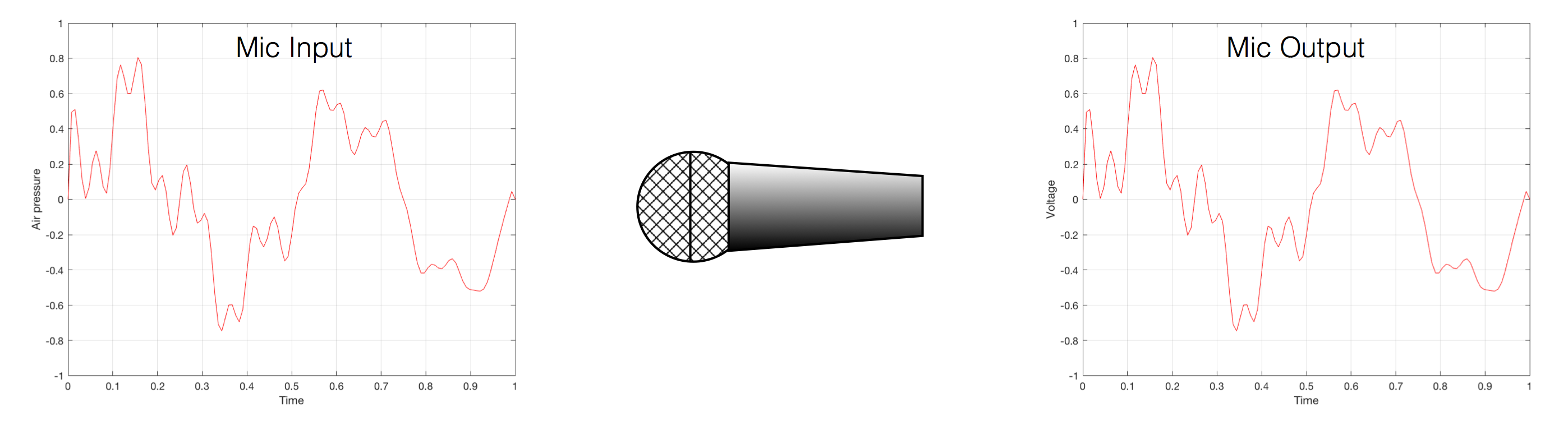Fig 1. Notice that (in theory, and ignoring a lot of things…) the change in air pressure over time at the input of the microphone is identical to the change in voltage over time at its output. Of course, this is not true in real life – microphones lie like a cheap rug…

At this point in the conversation, I’ll make a point to say that, in theory, we could “zoom in” on either of those two curves shown in Figure 1 and see more and more details. This is like looking at a map of Canada – it has lots of crinkly, jagged lines. If you zoom in and look at  the map of Newfoundland and Labrador, you’ll see that it has finer, crinkly, jagged lines. If you zoom in further, and stand where the water meets the shore in Trepassey and take a photo of your feet, you could copy it to draw a map of the line of where the water comes in around the rocks – and your toes – and you would wind up with even finer, crinkly, jagged lines… You could take this even further and get down to a microscopic or molecular level – but you get the idea… The point is that, in theory, both of the plots in Figure 1 have infinite resolution, both in time and in air pressure or voltage.

Now, let’s say that you wanted to take that microphone’s output and transmit it through a bunch of devices and wires that, in theory, all do nothing to the signal. Let’s say, for example, that you take the mic’s output, send it through a wire to a box that makes the signal twice as loud. Then take the output of that box and send it through a wire to another box that makes it half as loud. You take the output of that box and send it through a wire to a measuring device. What will you see? Unfortunately, none of the wires or boxes in the chain can be perfect, so you’ll probably see the signal plus something else which we’ll call the “error” in the system’s output. We can call it the error because, if we measure the input voltage and the output voltage at any one instant, we’ll probably see that they’re not identical. Since they should be identical, then the system must be making a mistake in transmitting the signal – so it makes errors…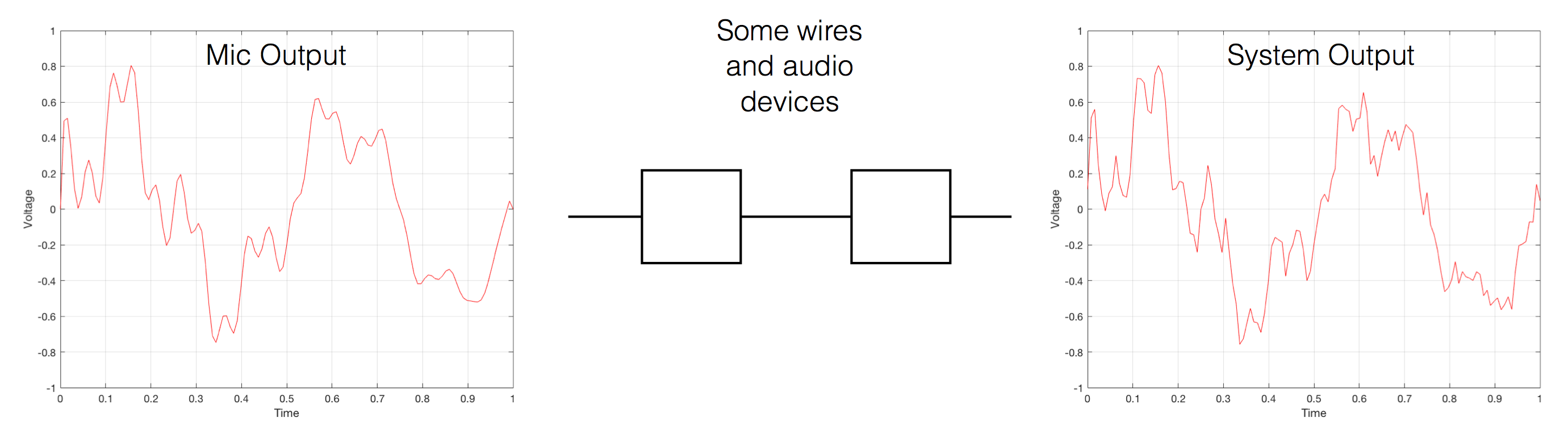Fig 2. If you send an audio signal through some wires and devices that (in theory) do nothing to the signal, you’ll find out that they add some extra stuff that you don’t want.

Pedantic Sidebar: Some people will call that error that the system adds to the signal “noise” – but I’m not going to call it that. This is because “noise” is a specific thing – noise is random – so if it’s not random, it’s not noise. Also, although the signal has been distorted (in that the output of the system is not identical to the input) I won’t call it “distortion” either, since distortion is a name that’s given to something that happens to the signal because the signal is there. (We would probably get at least some of the error out of our system even if we didn’t send any audio into it.) So, we could be slightly geeky and adequately vague and call the extra stuff “Distortion plus noise” but not “THD+N” – which stands for “Total Harmonic Distortion Plus Noise” – because not all kinds of distortion will produce a harmonic of the signal… but I’m getting ahead of myself…

So, we want to transmit (or store) the audio signal – but we want to reduce the noise caused by the transmission (or storage) system. One way to do this is to spend more money on your system. Use wires with better shielding, amplifiers with lower noise floors, bigger power supplies so that you don’t come close to their limits, run your magnetic tape twice as fast, and so on and so on. Or, you could convert the analogue signal (remember that it’s analogous to the change in air pressure over time) to one that is represented (and therefore transmitted or stored) digitally instead.

What does this mean?

## Conversion from analogue to digital and back(but skipping important details)

IMPORTANT: If you read this section, then please read the following postings as well. This is because, in order to keep things simple to start, I’m about to leave out some important details that I’ll add afterwards. However, if you don’t add the details, you could (understandably) jump to some incorrect conclusions (that many others before you have concluded…) So, if you don’t have time to read both sections, please don’t read either of them.

In the example above, we made a varying voltage that was analogous to the varying air pressure. If we wanted to store this, we could do it by varying the amount of magnetism on a wire or a coating on a tape, for example. Or we could cut a wiggly groove in a bit of vinyl that has a similar shape to the curve in the plots in Figure 1. Or, we could do something else: we could get a metronome (or a clock) and make a measurement of the voltage every time the metronome clicks, and write down the measurements.

For example, let’s zoom in on the first little bit of the signal in the plots in Figure 1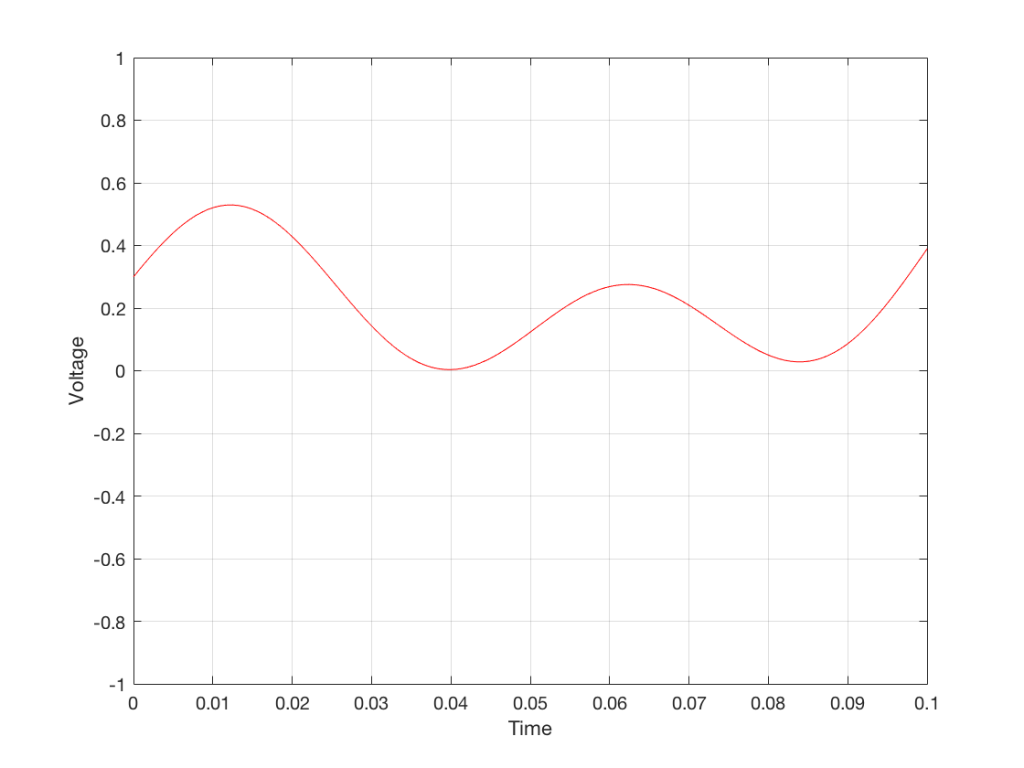Fig. 3 The same curve as was shown in Figure 1 – but zoomed in to the very beginning.

We’ll then put on a metronome and make a measurement of the voltage every time we hear the metronome click…

We can then keep the measurements (remembering how often we made them…) and write them down like this:

0.3000
0.4950
0.5089
0.3351
0.1116
0.0043
0.0678
0.2081
0.2754
0.2042
0.0730
0.0345
0.1775

We can store this series of numbers on a computer’s hard disk, for example. We can then come back tomorrow, and convert the measurements to voltages. First we read the measurements, and create the appropriate voltage…

We then make a “staircase” waveform by “holding” those voltages until the next value comes in.

All we need to do then is to use a low-pass filter to smooth out the hard edges of the staircase.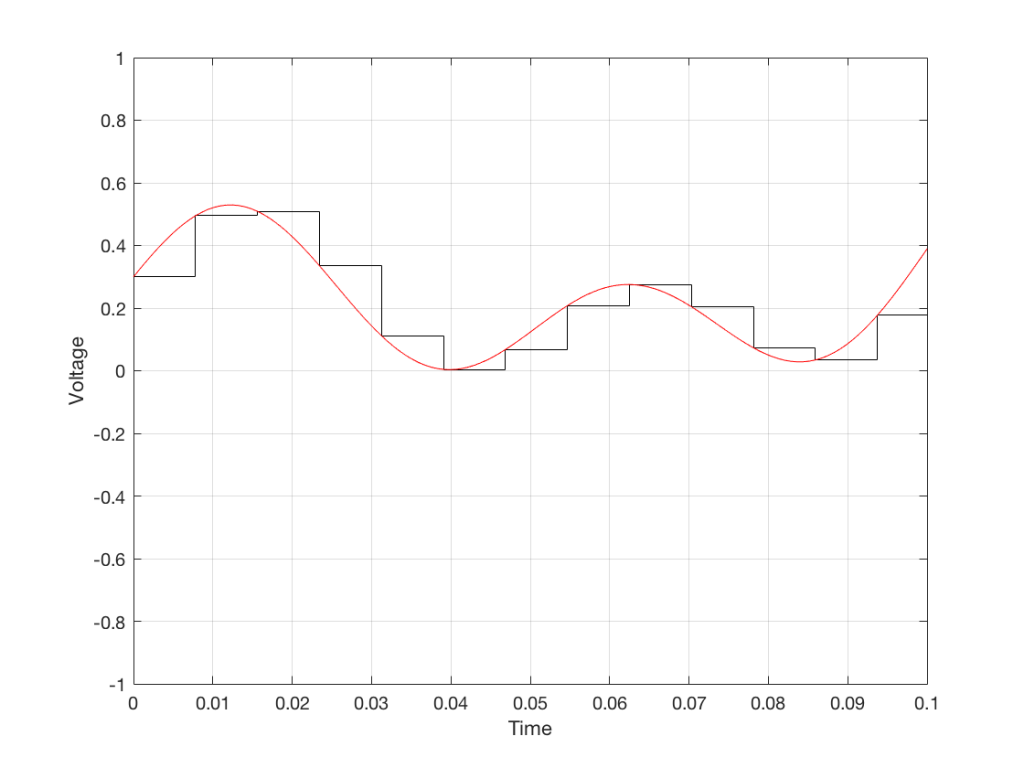Fig 7. When we smooth out the staircase, we get back the original signal (in red).

So, in this example, we’ve gone from an analogue signal (the red curve in Figure 3) to a digital signal (the series of numbers), and back to an analogue signal (the red curve in Figure 7).

In some ways, this is a bit like the way a movie works. When you watch a movie, you see a series of still photographs, probably taken at a rate of 24 pictures (or frames) per second. If you play those photos back at the same rate (24 fps or frames per second), you think you see movement. However, this is because your eyes and brain aren’t fast enough to see 24 individual photos per second – so you are fooled into thinking that things on the screen are moving.

However, digital audio is slightly different from film in two ways:

• The sound (equivalent to the movement in the film) is actually happening. It’s not a trick that relies on your ears and brain being too slow.
• If, when you were filming the movie, something were to happen between frames (say, the flash of a gunshot, for example) then it would never be caught on film. This is because the photos are discrete moments in time – and what happens between them is lost. However, if something were to make a very, very short sound between two samples (two measurements) in the digital audio signal – it would not be lost. This is because of something that happens at the beginning of the chain that I haven’t described… yet…

However, there are some “artefacts” (a fancy term for “weird errors”) that are present both in film and in digital audio that we should talk about.

The first is an error that happens when you mess around with the rate at which you take the measurements (called the “sampling rate”) or the photos (called the “frame rate”) – and, more importantly, when you need to worry about this. Let’s say that you make a film at 24 fps. If you play this back at a higher frame rate, then things will move very quickly (like old-fashioned baseball movies…). If you play them back at a lower frame rate, then things move in slow motion. So, for things to look “normal” you have to play the movie at the same rate that it was filmed. However, as long as no one is looking, you can transfer the movie as fast as you like. For example, if you wanted to copy the film, you could set up a movie camera so it was pointing at a movie screen and film the film. As long as the movie on the screen is running in sync with the camera, you can do this at any frame rate you like. But you’ll have to watch the copy at the same frame rate as the original film… (Note that this issue is not something that will come up in this series of postings about high resolution audio)

The second is an easy artefact to recognise. If you see a car accelerating from 0 to something fast on film, you’ll see the wheels of the car start to get faster and faster, then, as the car gets faster, the wheels slow down, stop, and then start going backwards… This does not happen in real life (unless you’re in a place lit with flashing lights like fluorescent bulbs or LED’s). I’ll do a posting explaining why this happens – but the thing to remember here is that the speed of the wheel rotation that you see on the film (the one that’s actually captured by the filming…) is not the real rotational speed of the wheel. However, those two rotational speeds are related to each other (and to the frame rate of the film). If you change the real rotational rate or the frame rate, you’ll change the rotational rate in the film. So, we call this effect “aliasing” because it’s a false version (an alias) of the real thing – but it’s always the same alias (assuming you repeat the conditions…) Digital audio can also suffer from aliasing, but in this case, you put in one frequency (which is actually the same as a rotational speed) and you get out another one. This is not the same as harmonic distortion, since the frequency that you get out is due to a relationship between the original frequency and the sampling rate, so the result is almost never a multiple of the input frequency. (We’re going to dig into this a lot deeper through this series of postings about high resolution audio, so if it doesn’t immediately make sense, don’t worry…)

## Some important details that I left out…

One of the things I said above was something like “we measure the voltage and store the results” and the example I gave was a nice series of numbers that only had 4 digits after the decimal point. This statement has some implications that we need to discuss.

Let’s say that I have a thing that I need to measure. For example, Figure 8 shows a piece of metal, and I want to measure its width.

Using my ruler, I can see that this piece of metal is about 57 mm wide. However, if I were geeky (and I am) I would say that this is not precise enough – and therefore it’s not accurate. The problem is that my ruler is only graduated in millimetres. So, if I try to measure anything that is not exactly an integer number of mm long, I’ll either have to guess (and be wrong) or round the measurement to the nearest millimetre (and be wrong).

So, if I wanted you to make a piece of metal the same width as my piece of metal, and I used the ruler in Figure 8, we would probably wind up with metal pieces of two different widths. In order to make this better, we need a better ruler – like the one in Figure 9.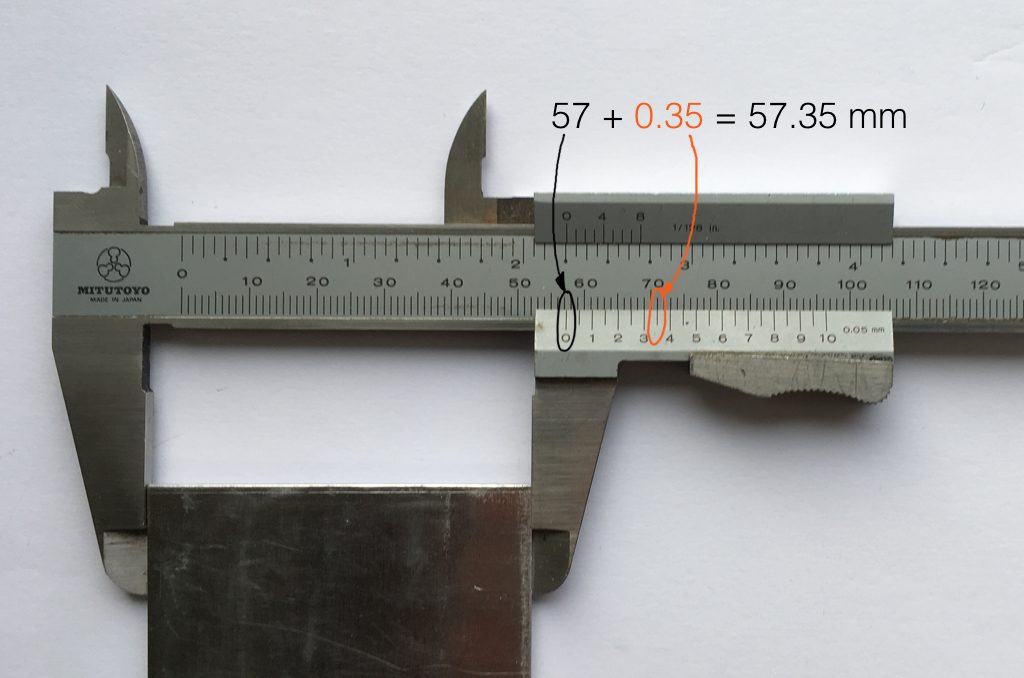Fig 9. The same piece of metal being measured with a vernier caliper. This gives us additional precision (down to 0.05 mm) so we can make a more accurate measurement.

Figure 9 shows a vernier caliper (a fancy type of ruler) being used to measure the same piece of metal. The caliper has a resolution of 0.05 mm instead of the 1 mm available on the ruler in Figure 8. So, we can make a much more accurate measurement of the metal because we have a measuring device with a higher precision.

The conversion of a digital audio signal is the same. As I said above, we measure the voltage of the electrical signal, and transmit (or store) the measurement. The question is: how accurate and precise is your measurement? As we saw above, this is (partly) determined by how many digits are in the number that you use when you “write down” the measurement.

Since the voltage measurements in digital audio are recorded in binary rather than decimal (we use 0 and 1 to write down the number instead of 0 up to 9) then we use Binary digITS – or “bits” instead of decimal digits (which are not called “dits”). The number of bits we have in the number that we write down (partly) determines the precision of the measurement of the voltage – and therefore (possibly), our accuracy…

Just like the example of the ruler in Figure 8, above, we have a limited resolution in our measurement. For example, if we had only 4 bits to work with then the waveform in 4 – the one we have to measure – would be measured with the “ruler” shown on the left side of Figure 10, below.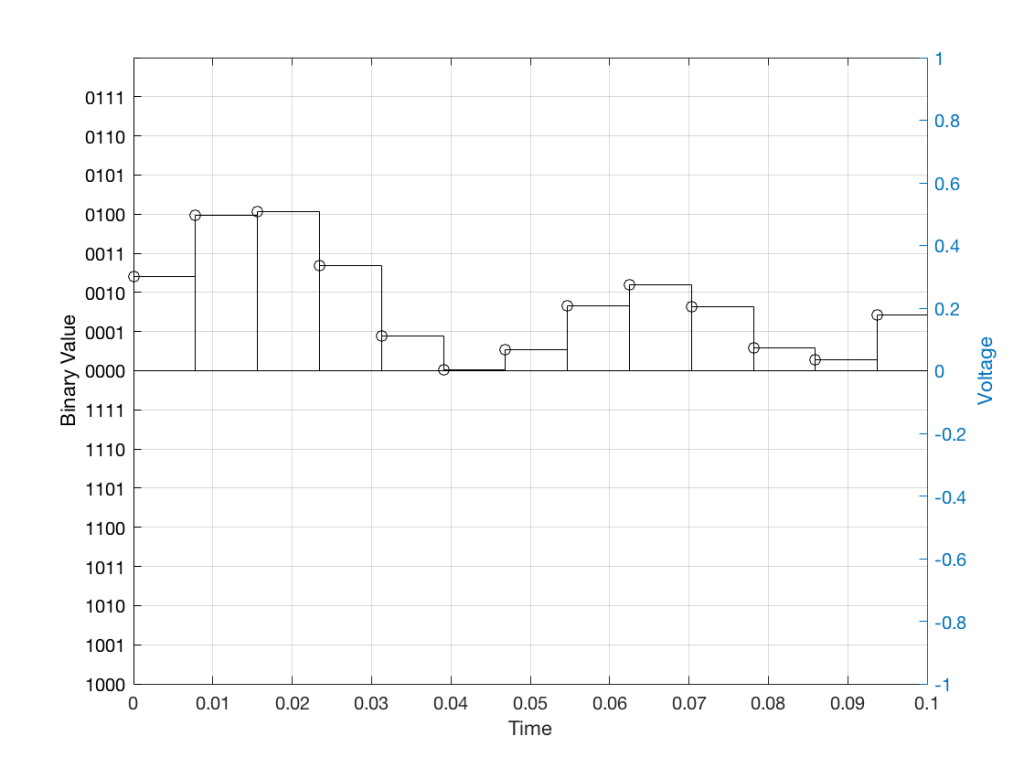Fig 10: The waveform from Figure 4 as a voltage (notice the Y-axis on the right). We have to measure these values using the ruler with the resolution shown on the Y-axis on the left.

When we do this, we have to round off the value to the nearest “tick” on our ruler, as shown in Figure 11.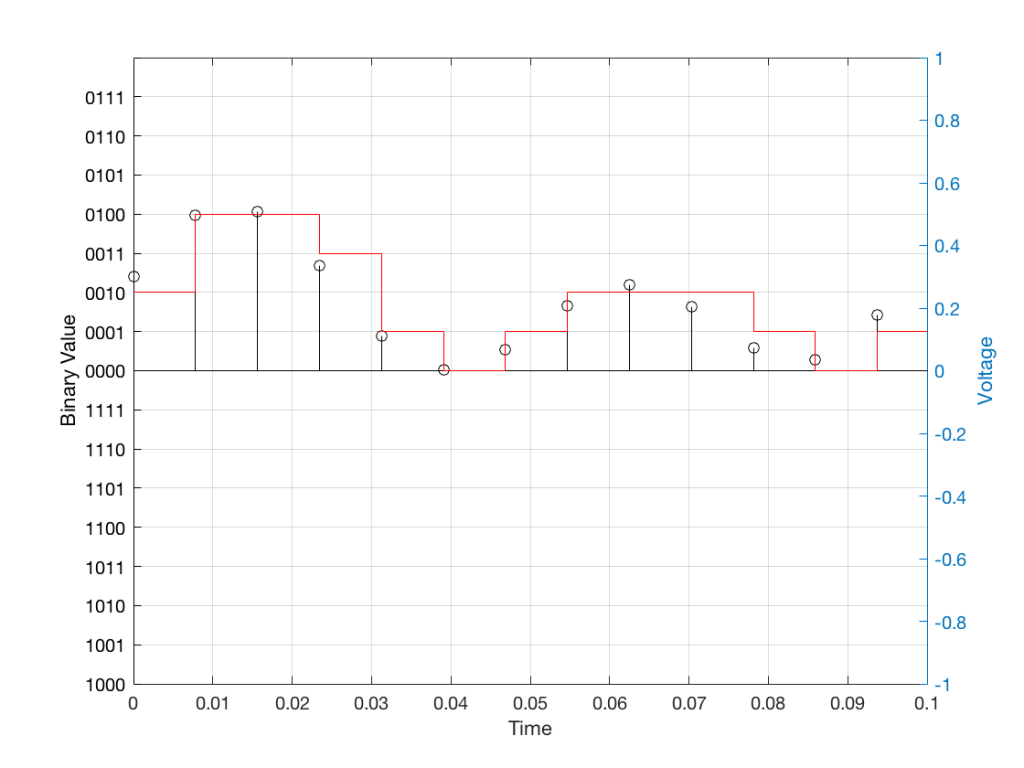Fig 11: The values from figure 10 (shown as the circles) rounded off to the nearest value on our 4-bit ruler (the red staircase).

Using this “ruler” which gives a write-down-able “quantity” to the measurement, we get the following values for the red staircase:

0010
0100
0100
0011
0001
0000
0001
0010
0010
0010
0001
0000
0001

When we “play these back” we get the staircase again, shown in Figure 12.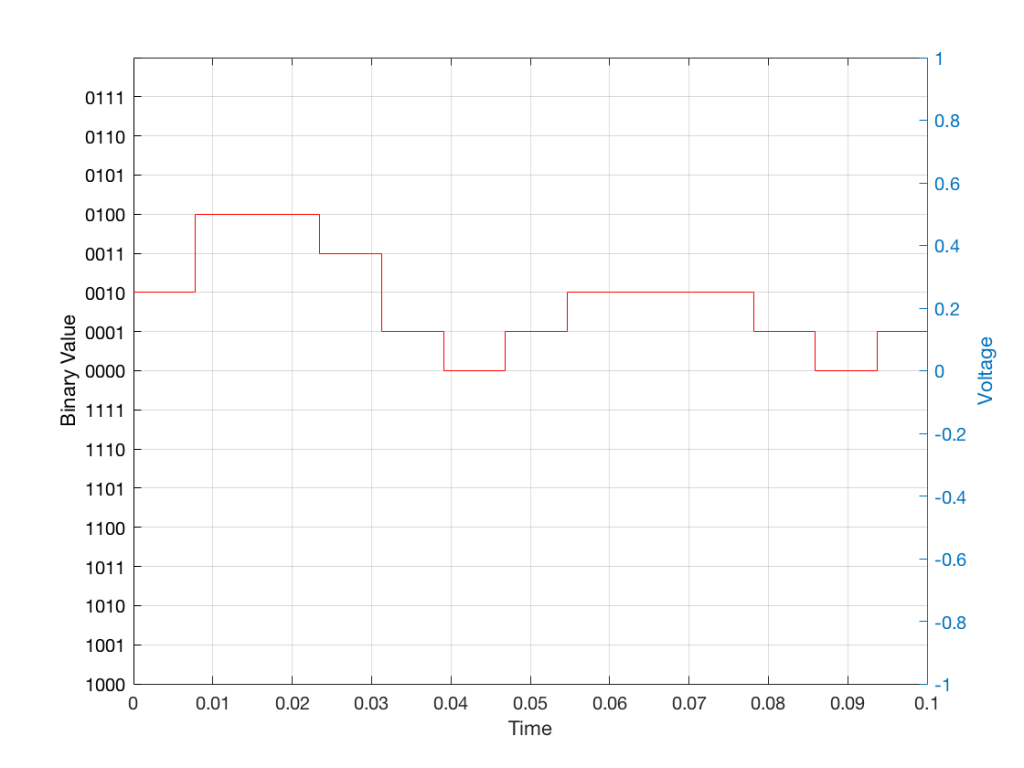Fig 12: The output of the measurements. Notice that all values sit exactly on one of the values for the “ruler” on the left Y-axis of the plot.

Of course, this means that, by rounding off the values, we have introduced an error in the system (just like the measurement in Figure 8 has a bigger error than the one in Figure 9). We can calculate this error if we just subtract the original signal from the output signal (in other words, Figure 12 minus Figure 10) to get Figure 13.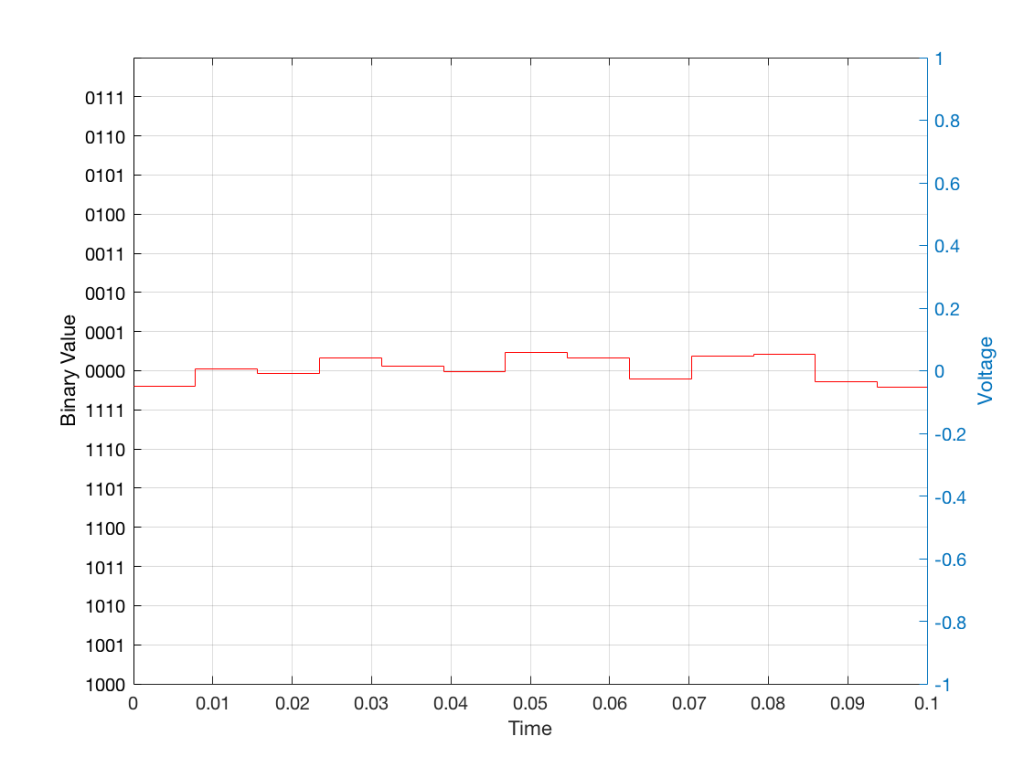Fig 13: The error that we produced due to the rounding off of the signal when we did the measurements. Notice that the error is always less than 0.5 of a “tick” of the ruler on the left Y-axis.

In order to improve our accuracy of the measurement, we have to increase the precision of the values. We can do this by adding an extra digit (or bit) to the number that we use to record the value.

If we were using decimal numbers (0-9) then adding an extra digit to the number would give us 10 times as many possibilities. (For example, if we were using 4 digits after the decimal in the example at the start of this posting, we have a total of 10,000 possible values – 0.0000 to 0.9999. If we add one more digit, we increase the resolution to 100,000 possible values – 0.00000 to 0.99999 ).

In binary, adding one extra digit gives us twice as many “ticks” on the ruler. So, using 4 bits gives us 16 possible values. Increasing to 5 bits gives us 32 possible values.

If you’re listening to a CD, then the individual measurements of each voltage – the “sample values” – are stored with 16 bits, which means that we have 65,536 possible values to pick from.

Remember that this means that we have more “ticks” on our ruler – but we don’t necessarily increase its range. So, for example, we’re still measuring a voltage from -1 V to 1 V – we just have more and more resolution with which we can do that measurement.

On to Part 2…

This is a good lecture from people-who-know-things about the difficulties in doing and interpreting headphone measurements.

# Translating Q to Q

As I’ve talked about in a previous posting, when a reciprocal peak/dip filter says “Q”, there’s no knowing what it might mean, because there are at least 7 different definitions of Q (3 for boosts and 4 for dips).

For many people, this doesn’t really matter. If you’re just playing with an EQ to make things sound better right now, then the values on the display really don’t matter: it’s the sound that counts.

If you’re like me, you need to be able to navigate between different pieces of software and hardware, and to get the same EQ response from them, then you’ll also need to know firstly that you can’t trust the display, and secondly, how to “translate” from device to device when necessary.

For example, take a look at Figure 1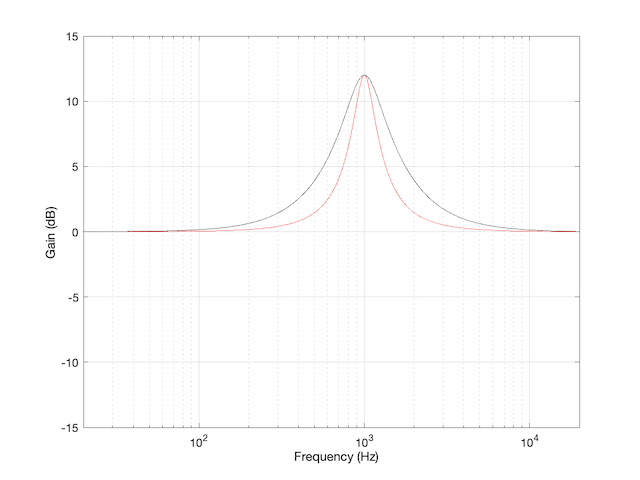Figure 1: The magnitude response of two peaking filters, both with Fc=1 kHz, Gain = +12 dB, Q = 2

This shows two magnitude responses, however, these are the measurements of two equalisers with identical settings:
Fc = 1 kHz, Gain = +12 dB, Q = 2.

The black curve shows the response of an equaliser that uses the -3 dB points to define the bandwidth of the filter, and therefore the Q is based on 1/(2 zeta). The red curve shows the response of an equaliser that uses the mid-point (in this case, +6 dB because the Gain is +12 dB) to define the bandwidth of the filter.

The difference between these two plots is shown below in Figure 2.

We’d have a similar problem if we were cutting instead of boosting, as shown in Figure 3.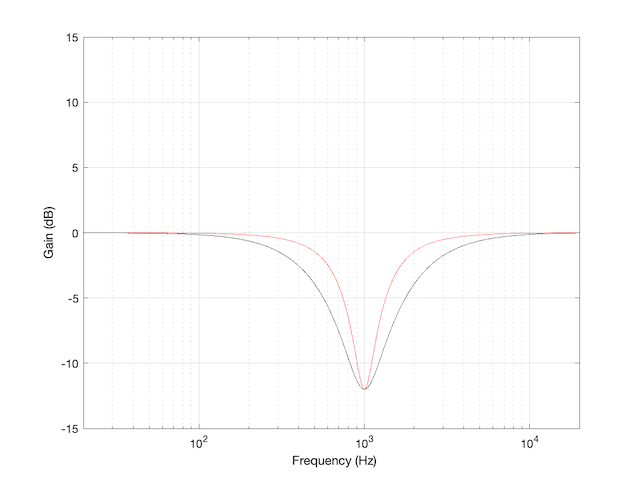Figure 3: The magnitude response of two peaking filters, both with Fc=1 kHz, Gain = -12 dB, Q = 2

You have to think upside down in this case, because the 1/(2 zeta) filter is actually using the 3 dB UP points to measure bandwidth; but we’ll ignore that and move on.

If you need to translate between the two systems shown above, there’s a pretty easy way to do it.

I’ll assume that you are implementing your filter using the mid-point definition of the bandwidth, so you need to convert into that system rather than out of it. (I’m making this assumption because it’s the one that Robert Bristow-Johnson used in his Audio Cookbook, which was freely copy-and-pasteable, which means that you find it everywhere these days.) Get the parameters from the filter you want to copy.

We’ll call these parameters Fc (for centre frequency, in Hz),(Gain in dB), and. I’m calling itbecause it’s a Q based on 1/(2 zeta) and we’ll need to keep it separate from our other Q, which I’ll call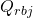(for Robert Bristow-Johnson).

Convert the gain into linear.Then do the following:

IF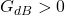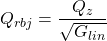ELSEIFELSE
your filter isn’t doing anything because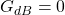END

## Example 1

If you have a -3 dB-based filter with the following parameters: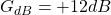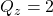and you want to implement that using the Bristow-Johnson equations, then you’ll have to use the following parameters: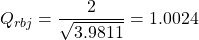## Example 2

If you have a -3 dB-based filter with the following parameters: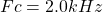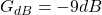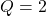and you want to implement that using the Bristow-Johnson equations, then you’ll have to use the following parameters:## Two Extra Things…

If the filter that you’re translating FROM is based on Andy Moorer’s design (which is based on the gain mid-point if the gain is within the ±6 dB range, but based on the 3 dB points if it’s outside that), then you’ll have to write your own IF/THEN statements.

If you’re implementing a filter that was specified for RBJ’s equations in a system that’s based on 1/(2 zeta), then you’re probably smart enough to figure out how to do the above in reverse.